# JP2009188748A - Inverter circuit, shift register circuit, nor circuit, and nand circuit - Google Patents

Inverter circuit, shift register circuit, nor circuit, and nand circuit Download PDF

## Info

Publication number
JP2009188748A
JP2009188748A JP2008026741A JP2008026741A JP2009188748A JP 2009188748 A JP2009188748 A JP 2009188748A JP 2008026741 A JP2008026741 A JP 2008026741A JP 2008026741 A JP2008026741 A JP 2008026741A JP 2009188748 A JP2009188748 A JP 2009188748A
Authority
JP
Japan
Prior art keywords
output transistor
transistor
output
source
drain region
Prior art date
Legal status (The legal status is an assumption and is not a legal conclusion. Google has not performed a legal analysis and makes no representation as to the accuracy of the status listed.)
Pending
Application number
JP2008026741A
Other languages
Japanese (ja)
Inventor
Seiichiro Jinda

Original Assignee
Sony Corp
ソニー株式会社
Priority date (The priority date is an assumption and is not a legal conclusion. Google has not performed a legal analysis and makes no representation as to the accuracy of the date listed.)
Filing date
Publication date
Application filed by Sony Corp, ソニー株式会社 filed Critical Sony Corp
Priority to JP2008026741A priority Critical patent/JP2009188748A/en
Publication of JP2009188748A publication Critical patent/JP2009188748A/en
Application status is Pending legal-status Critical

## Images

•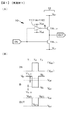•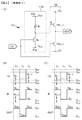•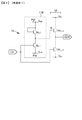•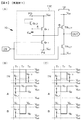••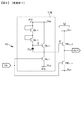•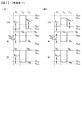•••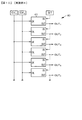•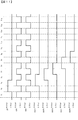•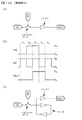•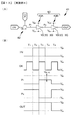•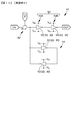•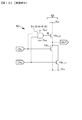••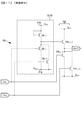•••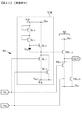•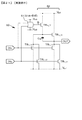•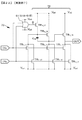•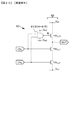•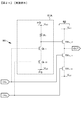•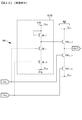•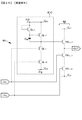•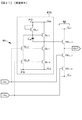•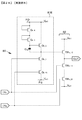•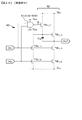•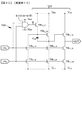•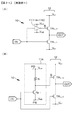••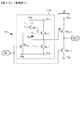•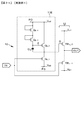•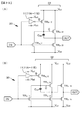•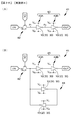•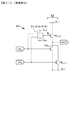•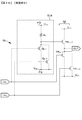••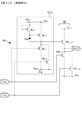•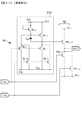•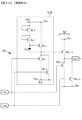••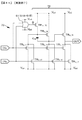•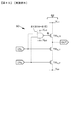•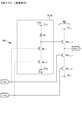••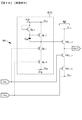•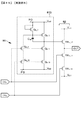•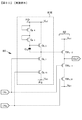•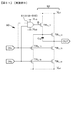•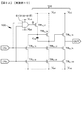••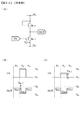•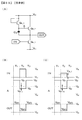## Abstract

An inverter circuit including transistors of the same conductivity type is provided.
An inverter circuit includes a negative logic configuration unit and an output circuit unit, and the output circuit unit includes two transistors of the same conductivity type. When expressed as a first output transistor and a second output transistor, one source / drain region of the first output transistor and one source / drain region of the second output transistor are connected to each other, and The gate electrode is connected to the output side of the negative logic component, the first voltage is applied to the other source / drain region of the first output transistor, and the other source / drain region of the second output transistor is applied to the other source / drain region. A second voltage is applied, and an input signal is applied to the gate electrode of the first output transistor and the input side of the negative logic component.
[Selection] Figure 1

## Description

The present invention relates to an inverter circuit, a shift register circuit, a negative logical sum (NOR) circuit, and a negative logical product (NAND) circuit, and more particularly to a circuit composed of transistors of the same conductivity type.

An inverter circuit is widely used as a circuit constituting a drive circuit or the like in a display device, a semiconductor memory device, or the like.

The inverter circuit can be formed by combining, for example, an n-channel transistor and a p-channel transistor. However, when a combination of transistors having different conductivity types is used, a manufacturing process of a p-channel transistor and an n-channel transistor is necessary. Therefore, when an inverter circuit is formed using only transistors of the same conductivity type (for example, only n-channel transistors) as the transistors, the manufacturing process can be reduced and productivity and yield can be improved. . Conventionally, inverter circuits configured using transistors of the same conductivity type have been proposed.

FIG. 53A is a circuit diagram of a known inverter circuit configured by, for example, an n-channel transistor Q n_1 and a resistor R 1 . 53B and 53C are schematic timing charts for explaining the operation of the circuit shown in FIG. In this circuit, one source / drain region of the transistor Q n_1 and one end of the resistor R 1 are connected to form an output terminal. A voltage V ss (for example, 0 volt) is applied to one source / drain region of the transistor Q n_1 , and a voltage V dd (for example, 10 volt) is applied to the other end of the resistor. An input signal IN is applied to the gate electrode of the transistor Q n_1 . Note that the low level of the input signal IN is the voltage V ss and the high level is the voltage V dd .

In a period T 1 shown in FIG. 53B, the input signal IN is at a low level, and the transistor Q n_1 is in an off state. The output voltage V OUT1 of the inverter circuit in the period T 1 is at a high level (V dd ). Since in the period T 2 is changed from the input signal IN is low level to a high level, the transistor Q n_1 is turned on from the off state. For this reason, the output of the inverter circuit goes from the high level to the low level. However, the value of the output voltage V OUT2 so determined by the voltage division ratio between the resistance value of the on value of the resistance and the resistance R 1 of the transistor Q n_1, the output voltage V OUT2 is not a critical low level. In the period T 3 , the input signal IN is at a low level, and the transistor Q n_1 is in an off state. The output voltage V OUT3 of the inverter circuit in the period T 3 is at a high level (V dd ).

In the circuit shown in FIG. 53A, the input signal IN is the gate-source voltage (V gs ) of the transistor Q n_1 . Even when the high level of the input signal IN does not reach the voltage Vdd , the inverter circuit shown in FIG. 53A operates. Specifically, as shown in FIG. 53C , when the value of the input signal IN exceeds the threshold voltage V th_1 of the transistor Q n_1 in the period T 2 , the output of the inverter circuit changes from the high level to the low level. Head. Therefore, the circuit shown in FIG. 53A also operates as a level shifter.

54A is a circuit diagram of an inverter circuit in which the resistor R 1 shown in FIG. 53A is replaced with a so-called diode-connected n-channel transistor Q n_2 . 54B and 54C are schematic timing charts for explaining the operation of the circuit shown in FIG. In this inverter circuit, the value of the output voltage V OUT2 is determined by the voltage division ratio between the resistance value of the on-resistance value and the transistor Q n_2 transistor Q n_1, the output voltage V OUT2 is not a critical low level. In the periods T 1 and T 3 , the output of the inverter circuit basically becomes high level. However, since the output value changes by the threshold voltage V th_2 of the transistor Q n_2 , the output voltages V OUT1 and V OUT3 do not become a complete high level. Similarly to the circuit shown in FIG. 53A, as shown in FIG. 54C , if the value of the input signal IN exceeds the threshold voltage V th_1 of the transistor Q n_1 in the period T 2 , The output of the inverter circuit goes from high level to low level. Therefore, like the circuit shown in FIG. 53A, the circuit shown in FIG. 54A also operates as a level shifter.

As described above, in the inverter circuit shown in FIG. 54A, the output voltages V OUT1 and V OUT3 do not become a complete high level (V dd ). A so-called bootstrap type inverter circuit has also been proposed as an inverter circuit that can solve this problem (see, for example, Non-Patent Document 1).

FIG. 55A is a circuit diagram of an inverter circuit using a so-called bootstrap operation. 55B and 55C are schematic timing charts for explaining the operation of the circuit shown in FIG. In this inverter circuit, the value of the output voltage V OUT2 is determined by the voltage division ratio between the resistance value of the on-resistance value and the transistor Q n_2 transistor Q n_1, the output voltage V OUT2 is not a critical low level. However, this inverter circuit is composed of three n-channel transistors Q n_1 , Q n_2 , and Q n_3 , and the output can be set to a complete high level (V dd ) in the periods T 1 and T 3 . One source / drain region of the transistor Q n_1 is connected to one source / drain region of the transistor Q n_2 to form an output terminal. The gate electrode of the transistor Q n_2 is connected to one source / drain region of the transistor Q n_3, configure the node A. When the transistor Q n — 3 is turned off from the on state, the node A is in a floating state. The gate electrode of the transistor Q n_3 is connected to the other source / drain region of the transistor Q n_3. A voltage V ss is applied to the other source / drain region of the transistor Q n_1 . A voltage V dd is applied to the other source / drain region of the transistor Q n_2, the voltage V dd is applied to the other source / drain region of the transistor Q n_3. An input signal IN is applied to the gate electrode of the transistor Q n_1 .

Incidentally, between the gate electrode and one of the source / drain region of the transistor Q n_2, or between the gate electrode and the other source / drain region of the transistor Q n_2 or capacitive portion of the bootstrap capacitor to both these, Sometimes connected. In the circuit shown in FIG. 55A , a capacitor part C ap as a bootstrap capacitor is connected between the gate electrode of the transistor Q n_2 and one of the source / drain regions. The capacitor part C ap can be constituted by, for example, a conductive layer sandwiching an insulating layer, or can be constituted as a so-called MOS capacitor part.

When the input signal IN changes from the low level to the high level, the transistor Q n_1 is turned from the off state to the on state, and the output voltage V OUT2 goes to the low level. Along with this, the potential of the node A also decreases. When the potential difference between the gate electrode of the node A and the transistor Q n_3 reaches the threshold voltage V Th_3 transistor Q n_3, transistor Q n_3 is turned off. Therefore, as illustrated in FIG. 55B, the potential V A2 of the node A in the period T 2 is (V dd −V th — 3 ), and the node A is in a floating state. When the input signal IN is changed from a high level to a low level in the period T 3 , the transistor Q n_1 is changed from an on state to an off state, and the output voltage of the inverter circuit is changed from a low level to a high level. Since the node A is in a floating state, the potential of the node A rises following the output voltage of the inverter circuit, and the potential V A3 in the period T 3 exceeds the high level (V dd ). If the value of (V A3 −V dd ) is set to exceed the value of the threshold voltage V th_2 of the transistor Q n_2 , the output voltage V OUT3 of the inverter circuit is completely high level (V dd) in the period T 3 . ). As shown in FIG. 55C , when the value of the input signal IN exceeds the threshold voltage V th_1 of the transistor Q n_1 in the period T 2 , the output of the inverter circuit goes from the high level to the low level. Therefore, like the circuits shown in FIGS. 53A and 54A, the circuit shown in FIG. 55A also operates as a level shifter.

Hara, "Basics of MOS Integrated Circuits", Modern Science, p. 94-96

As described above, the inverter circuit using the bootstrap operation shown in FIG. 55A cannot output a complete low level and a complete high level. In an inverter circuit using only transistors of the same conductivity type as a transistor, there is a problem that the width of the maximum amplitude of output is narrowed. As one method, the inventors have proposed a bootstrap circuit in which a reset transistor and a precharge transistor are added in Japanese Patent Application Laid-Open No. 2005-143068. However, it is undeniable that the circuit structure and its control become complicated, for example, a control pulse signal for a reset / precharge transistor synchronized with the input signal is required in addition to the input signal.

Accordingly, an object of the present invention is to provide an inverter circuit composed of transistors of the same conductivity type, which can output a complete low level and a complete high level without requiring a control pulse signal different from an input signal, and A shift register circuit, a negative logical product circuit, and a negative logical sum circuit including the inverter circuit are provided.

An inverter circuit of the present invention for achieving the above object is a shift register circuit composed of transistors of the same conductivity type,
The inverter circuit consists of a negative logic component and an output circuit,
The output circuit section is composed of two transistors of the same conductivity type.
When the two transistors constituting the output circuit unit are expressed as a first output transistor and a second output transistor,
One source / drain region of the first output transistor and one source / drain region of the second output transistor are connected,
The gate electrode of the second output transistor is connected to the output side of the negative logic component,
A first voltage is applied to the other source / drain region of the first output transistor, a second voltage is applied to the other source / drain region of the second output transistor,
An input signal is applied to the gate electrode of the first output transistor and the input side of the negative logic component.

In the inverter circuit of the present invention,
When an input signal for turning off the first output transistor is applied, a voltage sufficient to maintain the on state of the second output transistor from the output side of the negative logic component is applied to the gate electrode of the second output transistor. Applied,
When an input signal for turning on the first output transistor is applied, a voltage sufficient to maintain the off state of the second output transistor from the output side of the negative logic component is applied to the gate electrode of the second output transistor. Applied,
The output signal can be output from a connection portion between one source / drain region of the first output transistor and one source / drain region of the second output transistor.

In the inverter circuit configured as described above, when an input signal for turning off the first output transistor is applied, the second output transistor is connected to the gate electrode of the second output transistor from the output side of the negative logic component. A voltage sufficient to maintain the ON state of is applied. Therefore, in this case, the second voltage is output as the output voltage. On the other hand, when an input signal for turning on the first output transistor is applied, the first voltage is output as the output voltage. Thereby, an output signal having the first voltage and the second voltage as output voltages can be output.

Alternatively, in the inverter circuit of the present invention,
The output circuit unit further includes two transistors of the same conductivity type, and when these two transistors are represented as a third output transistor and a fourth output transistor,
One source / drain region of the third output transistor and one source / drain region of the fourth output transistor are connected,
The gate electrode of the fourth output transistor is connected to one source / drain region of the first output transistor and one source / drain region of the second output transistor,
A first voltage is applied to the other source / drain region of the third output transistor, a second voltage is applied to the other source / drain region of the fourth output transistor,
An input signal is applied to the gate electrode of the third output transistor,
When an input signal that turns off the first output transistor and the third output transistor is applied, the gate electrode of the fourth output transistor has a voltage sufficient to maintain the on state of the fourth output transistor by the bootstrap operation. Is applied,
When an input signal for turning on the first output transistor and the third output transistor is applied, a voltage sufficient to maintain the off state of the fourth output transistor is applied to the gate electrode of the fourth output transistor,
The output signal may be output from a connection portion between one source / drain region of the third output transistor and one source / drain region of the fourth output transistor.

In the inverter circuit having the above-described configuration, when an input signal for turning off the first output transistor and the third output transistor is applied, the fourth output is applied to the gate electrode of the fourth output transistor by the bootstrap operation. A voltage is applied that is sufficient to maintain the transistor on. Therefore, in this case, the second voltage is output as the output voltage. On the other hand, when an input signal for turning on the first output transistor and the third output transistor is applied, the first voltage is output as the output voltage. Thereby, an output signal having the first voltage and the second voltage as output voltages can be output. In this configuration, it is not necessary to apply a voltage sufficient to maintain the ON state of the second output transistor from the output side of the negative logic configuration unit. Therefore, there is an advantage that the setting condition of the voltage for operating the negative logic component is relaxed and suitable for common use of the power supply voltage.

In the inverter circuit having the above-described third output transistor and fourth output transistor,
The output circuit unit further includes two transistors of the same conductivity type, and when these two transistors are represented as a fifth output transistor and a sixth output transistor,
One source / drain region of the fifth output transistor and one source / drain region of the sixth output transistor are connected,
The gate electrode of the sixth output transistor is connected to one source / drain region of the third output transistor and one source / drain region of the fourth output transistor,
A third voltage is applied to the other source / drain region of the fifth output transistor, a fourth voltage is applied to the other source / drain region of the sixth output transistor,
An input signal is applied to the gate electrode of the fifth output transistor,
When an input signal that turns off the first output transistor, the third output transistor, and the fifth output transistor is applied, the gate electrode of the sixth output transistor has one source / drain region of the third output transistor. And a voltage sufficient to maintain the on state of the sixth output transistor is applied from the connection between the source / drain region of the fourth output transistor and the sixth output transistor,
When an input signal for turning on the first output transistor, the third output transistor, and the fifth output transistor is applied, the gate electrode of the sixth output transistor has one source / drain region of the third output transistor. And a voltage sufficient to maintain the off state of the sixth output transistor from the connection between the source / drain region of the fourth output transistor and the sixth output transistor,
The output signal can be output from a connection portion between one source / drain region of the fifth output transistor and one source / drain region of the sixth output transistor.

In the inverter circuit having the above-described configuration, when an input signal for turning off the first output transistor, the third output transistor, and the fifth output transistor is applied, the gate electrode of the sixth output transistor A voltage sufficient to maintain the on state of the sixth output transistor is applied from a connection portion between one source / drain region of the third output transistor and one source / drain region of the fourth output transistor. Therefore, in this case, the fourth voltage is output as the output voltage. On the other hand, when an input signal for turning on the first output transistor, the third output transistor, and the fifth output transistor is applied, the third voltage is output as the output voltage. By setting the width between the third voltage and the fourth voltage to be smaller than the width between the first voltage and the second voltage, the reliability in the operation of the inverter circuit can be improved.

In the inverter circuit of the present invention including the above-described various preferred configurations (hereinafter sometimes simply referred to as the inverter circuit of the present invention), the negative logic component is composed of transistors of the same conductivity type and resistors. Configured,
The gate electrode of the transistor constitutes the input side of the negative logic component,
One source / drain region of the transistor is connected to one end of the resistor and constitutes the output side of the negative logic component,
The other end of the resistor is connected to one voltage supply line,
The other source / drain region of the transistor can be connected to the other voltage supply line.

Alternatively, the negative logic component is composed of a first transistor and a second transistor of the same conductivity type,
The gate electrode of the first transistor constitutes the input side of the negative logic component,
One source / drain region of the first transistor is connected to one source / drain region of the second transistor, and constitutes an output side of the negative logic component,
The other source / drain region of the second transistor is connected to one voltage supply line,
The gate electrode of the second transistor is connected to the other source / drain region of the second transistor,
The other source / drain region of the first transistor can be connected to the other voltage supply line.

Alternatively, the negative logic component is composed of a first transistor, a second transistor, and a third transistor of the same conductivity type,
In the first transistor,
(A-1) The gate electrode constitutes the input side of the negative logic component,
(A-2) One source / drain region is connected to one source / drain region of the second transistor, and constitutes the output side of the negative logic component,
In the second transistor,
(B-1) The other source / drain region is connected to one voltage supply line,
(B-2) The gate electrode is connected to one source / drain region of the third transistor,
In the third transistor,
(C-1) The gate electrode is connected to the other source / drain region,
(C-2) The other source / drain region is connected to one voltage supply line,
The other source / drain region of the first transistor can be connected to the other voltage supply line.

Alternatively, the negative logic component is composed of a first transistor, a second transistor, and a third transistor of the same conductivity type,
In the first transistor,
(A-1) The gate electrode constitutes the input side of the negative logic component,
(A-2) One source / drain region is connected to one source / drain region of the second transistor, and constitutes the output side of the negative logic component,
In the second transistor,
(B-1) The other source / drain region is connected to one voltage supply line,
(B-2) The gate electrode is connected to one source / drain region of the third transistor,
In the third transistor,
(C-1) The gate electrode is connected to the other source / drain region,
The negative logic component further includes a fourth transistor of the same conductivity type,
The other source / drain region of the third transistor is connected to one voltage supply line,
One source / drain region of the fourth transistor is connected to the gate electrode of the second transistor and one source / drain region of the third transistor,
The other source / drain region of the first transistor and the other source / drain region of the fourth transistor are connected to the other voltage supply line,
An input signal can be applied to the gate electrode of the fourth transistor.

Alternatively, the negative logic component is composed of a first transistor, a second transistor, and a third transistor of the same conductivity type,
In the first transistor,
(A-1) The gate electrode constitutes the input side of the negative logic component,
(A-2) One source / drain region is connected to one source / drain region of the second transistor, and constitutes the output side of the negative logic component,
In the second transistor,
(B-1) The other source / drain region is connected to one voltage supply line,
(B-2) The gate electrode is connected to one source / drain region of the third transistor,
In the third transistor,
(C-1) The gate electrode is connected to the other source / drain region,
The negative logic component further includes a fourth transistor of the same conductivity type,
The other source / drain region of the third transistor is connected to one source / drain region of the fourth transistor,
The gate electrode of the fourth transistor is connected to the other source / drain region of the fourth transistor,
The other source / drain region of the fourth transistor is connected to one voltage supply line,
The other source / drain region of the first transistor can be connected to the other voltage supply line.

To achieve the above object, a shift register circuit of the present invention is a shift register circuit composed of transistors of the same conductivity type, and is a shift register circuit composed of transistors of the same conductivity type,
The shift register circuit is composed of a transfer gate unit and a buffer circuit,
The transfer gate part is composed of transistors of the same conductivity type.
(A-1) An input signal is applied to one source / drain region,
(A-2) A clock signal is applied to the gate electrode,
The buffer circuit is composed of a first inverter circuit portion and a second inverter circuit portion,
(B-1) The first inverter circuit portion is connected to the other source / drain region of the transistor constituting the transfer gate portion,
(B-2) The output signal of the first inverter circuit unit is applied as the input signal of the second inverter circuit unit,
(B-3) As an output signal of the buffer circuit, an output signal is output from the second inverter circuit unit.
The present invention relates to a shift register circuit.

In the shift register circuit of the present invention, the first inverter circuit section is composed of the inverter circuit of the present invention. That is, the first inverter circuit unit is an inverter circuit unit composed of transistors of the same conductivity type,
The first inverter circuit section is composed of a negative logic configuration section and an output circuit section,
The output circuit section is composed of two transistors of the same conductivity type.
When the two transistors constituting the output circuit unit are expressed as a first output transistor and a second output transistor,
One source / drain region of the first output transistor and one source / drain region of the second output transistor are connected,
The gate electrode of the second output transistor is connected to the output side of the negative logic component,
A first voltage is applied to the other source / drain region of the first output transistor, a second voltage is applied to the other source / drain region of the second output transistor,
An input signal is applied to the gate electrode of the first output transistor and the input side of the negative logic component according to the clock signal.

In the shift register circuit of the present invention, when an input signal for turning off the first output transistor is applied, the second output transistor is connected to the gate electrode of the second output transistor from the output side of the negative logic component. Sufficient voltage is applied to maintain the on state of
When an input signal for turning on the first output transistor is applied, a voltage sufficient to maintain the off state of the second output transistor from the output side of the negative logic component is applied to the gate electrode of the second output transistor. Applied,
The output signal of the first inverter circuit section can be output from a connection portion between one source / drain region of the first output transistor and one source / drain region of the second output transistor.

Alternatively, in the shift register circuit of the present invention, the output circuit unit further includes two transistors of the same conductivity type, and these two transistors are used as a third output transistor and a fourth output transistor. When
One source / drain region of the third output transistor and one source / drain region of the fourth output transistor are connected,
The gate electrode of the fourth output transistor is connected to one source / drain region of the first output transistor and one source / drain region of the second output transistor,
A first voltage is applied to the other source / drain region of the third output transistor, a second voltage is applied to the other source / drain region of the fourth output transistor,
An input signal is applied to the gate electrode of the third output transistor according to the clock signal,
When an input signal that turns off the first output transistor and the third output transistor is applied, the gate electrode of the fourth output transistor has a voltage sufficient to maintain the on state of the fourth output transistor by the bootstrap operation. Is applied,
When an input signal for turning on the first output transistor and the third output transistor is applied, a voltage sufficient to maintain the off state of the fourth output transistor is applied to the gate electrode of the fourth output transistor,
The output signal of the first inverter circuit section can be output from a connection portion between one source / drain region of the third output transistor and one source / drain region of the fourth output transistor.

In the shift register circuit of the present invention including the third output transistor and the fourth output transistor described above, the output circuit unit further includes two transistors of the same conductivity type. These two transistors Is expressed as a fifth output transistor and a sixth output transistor,
One source / drain region of the fifth output transistor and one source / drain region of the sixth output transistor are connected,
The gate electrode of the sixth output transistor is connected to one source / drain region of the third output transistor and one source / drain region of the fourth output transistor,
A third voltage is applied to the other source / drain region of the fifth output transistor, a fourth voltage is applied to the other source / drain region of the sixth output transistor,
An input signal is applied to the gate electrode of the fifth output transistor according to the clock signal,
When an input signal that turns off the first output transistor, the third output transistor, and the fifth output transistor is applied, the gate electrode of the sixth output transistor has one source / drain region of the third output transistor. And a voltage sufficient to maintain the on state of the sixth output transistor is applied from the connection between the source / drain region of the fourth output transistor and the sixth output transistor,
When an input signal for turning on the first output transistor, the third output transistor, and the fifth output transistor is applied, the gate electrode of the sixth output transistor has one source / drain region of the third output transistor. And a voltage sufficient to maintain the off state of the sixth output transistor from the connection between the source / drain region of the fourth output transistor and the sixth output transistor,
The output signal of the first inverter circuit unit can be output from a connection portion between one source / drain region of the fifth output transistor and one source / drain region of the sixth output transistor.

In the shift register circuit of the present invention, the transfer gate portion is also composed of transistors of the same conductivity type. For this reason, the amplitude of the potential on the input side of the first inverter circuit portion constituting the buffer circuit is reduced by the threshold voltage of the transistor constituting the transfer gate portion. However, for the same reason as described in the background art, the inverter circuit constituting the first inverter circuit unit also operates as a level shifter. Therefore, unless there is a problem that the threshold voltage of the transistor constituting the transfer gate portion is extremely large, the operation is not hindered.

In the shift register circuit of the present invention including the various preferable configurations described above, the inverter circuit constituting the second inverter circuit unit may be configured using the known inverter circuit described in the background art. From the viewpoint of widening the amplitude of the shift register circuit, the inverter circuit of the present invention described above is preferably used.

That is, the second inverter circuit unit is an inverter circuit unit composed of transistors of the same conductivity type,
The second inverter circuit part is composed of a negative logic component part and an output circuit part,
The output circuit part constituting the second inverter circuit part is composed of two transistors of the same conductivity type,
When the two transistors constituting the output circuit unit of the second inverter circuit unit are expressed as a first output transistor and a second output transistor,
One source / drain region of the first output transistor constituting the second inverter circuit portion and one source / drain region of the second output transistor constituting the second inverter circuit portion are connected,
The gate electrode of the second output transistor configuring the second inverter circuit unit is connected to the output side of the negative logic configuration unit configuring the second inverter circuit unit,
A first voltage is applied to the other source / drain region of the first output transistor that constitutes the second inverter circuit section, and a second voltage is applied to the other source / drain region of the second output transistor that constitutes the second inverter circuit section. 2 voltages are applied,
The output signal of the first inverter circuit unit is applied as an input signal to the gate electrode of the first output transistor configuring the second inverter circuit unit and the input side of the negative logic configuration unit configuring the second inverter circuit unit. It can be set as a structure.

In the second inverter circuit portion, when an input signal for turning off the first output transistor constituting the second inverter circuit portion is applied, the gate electrode of the second output transistor constituting the second inverter circuit portion Is applied with a voltage sufficient to maintain the ON state of the second output transistor constituting the second inverter circuit unit from the output side of the negative logic component constituting the second inverter circuit unit,
When an input signal for turning on the first output transistor constituting the second inverter circuit section is applied, the second inverter circuit section is formed on the gate electrode of the second output transistor constituting the second inverter circuit section. A voltage sufficient to maintain the OFF state of the second output transistor constituting the second inverter circuit unit is applied from the output side of the negative logic component unit,
The output signal of the second inverter circuit unit includes one source / drain region of the first output transistor constituting the second inverter circuit unit and one source / drain region of the second output transistor constituting the second inverter circuit unit. It can be set as the structure output from a connection part.

Alternatively, in the second inverter circuit unit, the output circuit unit constituting the second inverter circuit unit further includes two transistors of the same conductivity type, and these two transistors are connected to the third output transistor. , And the fourth output transistor,
One source / drain region of the third output transistor constituting the second inverter circuit portion and one source / drain region of the fourth output transistor constituting the second inverter circuit portion are connected,
The gate electrode of the fourth output transistor that constitutes the second inverter circuit portion is connected to one source / drain region of the first output transistor that constitutes the second inverter circuit portion and the second output transistor that constitutes the second inverter circuit portion. Connected to one of the source / drain regions,
The first voltage is applied to the other source / drain region of the third output transistor that constitutes the second inverter circuit portion, and the second source / drain region of the fourth output transistor that constitutes the second inverter circuit portion is the second source / drain region. 2 voltages are applied,
The output signal of the first inverter circuit unit is applied as an input signal to the gate electrode of the third output transistor constituting the second inverter circuit unit,
When an input signal for turning off the first output transistor constituting the second inverter circuit portion and the third output transistor constituting the second inverter circuit portion is applied, the fourth output transistor constituting the second inverter circuit portion A voltage sufficient to maintain the on state of the fourth output transistor that constitutes the second inverter circuit unit by the bootstrap operation is applied to the gate electrode of
When an input signal for turning on the first output transistor constituting the second inverter circuit portion and the third output transistor constituting the second inverter circuit portion is applied, the fourth output transistor constituting the second inverter circuit portion A voltage sufficient to maintain the OFF state of the fourth output transistor constituting the second inverter circuit section is applied to the gate electrode of
The output signal of the second inverter circuit unit includes one source / drain region of the third output transistor constituting the second inverter circuit unit and one source / drain region of the fourth output transistor constituting the second inverter circuit unit. It can be set as the structure output from a connection part.

In the second inverter circuit unit including the third output transistor and the fourth output transistor described above, the output circuit unit constituting the second inverter circuit unit further includes two transistors of the same conductivity type. When these two transistors are expressed as a fifth output transistor and a sixth output transistor,
The gate electrode of the sixth output transistor that constitutes the second inverter circuit portion is connected to one source / drain region of the third output transistor that constitutes the second inverter circuit portion and the fourth output transistor that constitutes the second inverter circuit portion. Connected to one of the source / drain regions,
The third voltage is applied to the other source / drain region of the fifth output transistor constituting the second inverter circuit portion, and the other source / drain region of the sixth output transistor constituting the second inverter circuit portion is the second source / drain region. 4 voltages are applied,
The output signal of the first inverter circuit part constituting the second inverter circuit part is applied as an input signal to the gate electrode of the fifth output transistor constituting the second inverter circuit part,
An input signal is applied to turn off the first output transistor constituting the second inverter circuit section, the third output transistor constituting the second inverter circuit section, and the fifth output transistor constituting the second inverter circuit section. The gate electrode of the sixth output transistor that constitutes the second inverter circuit portion has one source / drain region of the third output transistor that constitutes the second inverter circuit portion and the second inverter circuit portion that constitutes the second inverter circuit portion. A voltage sufficient to maintain the ON state of the sixth output transistor constituting the second inverter circuit section is applied from the connection portion of the four output transistors to one of the source / drain regions,
An input signal for turning on the first output transistor constituting the second inverter circuit unit, the third output transistor constituting the second inverter circuit unit, and the fifth output transistor constituting the second inverter circuit unit is applied. The gate electrode of the sixth output transistor that constitutes the second inverter circuit portion has one source / drain region of the third output transistor that constitutes the second inverter circuit portion and the second inverter circuit portion that constitutes the second inverter circuit portion. A voltage sufficient to maintain the off state of the sixth output transistor constituting the second inverter circuit portion is applied from the connection portion of one of the four output transistors to one of the source / drain regions,
The output signal of the second inverter circuit section includes one source / drain region of the fifth output transistor constituting the second inverter circuit section and one source / drain area of the sixth output transistor constituting the second inverter circuit section. It can be set as the structure output from a connection part.

In order to achieve the above object, a NOR circuit of the present invention is a NOR circuit composed of transistors of the same conductivity type, to which at least a first input signal and a second input signal are applied. And
It consists of a negative logical sum component and an output circuit unit,
The output circuit section is composed of two transistors of the same conductivity type.
When the two transistors constituting the output circuit unit are expressed as a first output transistor and a second output transistor,
One source / drain region of the first output transistor and one source / drain region of the second output transistor are connected,
The gate electrode of the second output transistor is connected to the output side of the NOR circuit,
The negative OR circuit further includes a transistor of the same conductivity type. In the transistor, one source / drain region includes one source / drain region of the first output transistor and the second output transistor. Connected to one source / drain region, a first voltage is applied to the other source / drain region, a second input signal is applied to the gate electrode,
A first voltage is applied to the other source / drain region of the first output transistor,
A second voltage is applied to the other source / drain region of the second output transistor,
A first input signal and a second input signal are applied to the negative logical sum component,
A first input signal is applied to the gate electrode of the first output transistor,
The output signal is output from a connection portion between one source / drain region of the first output transistor and one source / drain region of the second output transistor.

In order to achieve the above object, a NAND circuit of the present invention is a NAND circuit composed of transistors of the same conductivity type, to which at least a first input signal and a second input signal are applied. And
It consists of a negative AND component and an output circuit.
The output circuit section is composed of two transistors of the same conductivity type.
When the two transistors constituting the output circuit unit are expressed as a first output transistor and a second output transistor,
One source / drain region of the first output transistor and one source / drain region of the second output transistor are connected,
The gate electrode of the second output transistor is connected to the output side of the negative AND component,
The NAND circuit further includes a transistor of the same conductivity type, in which one source / drain region is connected to the other source / drain region of the first output transistor, and the other A first voltage is applied to the source / drain regions of the first electrode, a second input signal is applied to the gate electrode,
A second voltage is applied to the other source / drain region of the second output transistor,
A first input signal and a second input signal are applied to the negative AND component,
A first input signal is applied to the gate electrode of the first output transistor,
The output signal is output from a connection portion between one source / drain region of the first output transistor and one source / drain region of the second output transistor.

The inverter circuit of the present invention, the first inverter circuit section provided in the shift register circuit of the present invention, the second inverter circuit section provided in the shift register circuit of the present invention (the second inverter circuit section is composed of the inverter circuit of the present invention) In some cases, this is simply referred to as an inverter circuit of the present invention.

The inverter circuit of the present invention, the shift register circuit of the present invention, the NAND circuit of the present invention, and the NOR circuit of the present invention (hereinafter, these may be collectively referred to simply as the circuit of the present invention) , N-channel transistors may be used. Alternatively, it may be composed of a p-channel transistor. The transistor may be a thin film transistor (TFT) or a transistor formed on a semiconductor substrate or the like. The structure of the transistor is not particularly limited. In the following description, the transistor is described as an enhancement type, but is not limited thereto. If there is no problem in operation, a depletion type transistor can be used. Further, the transistor may be a single gate type or a dual gate type.

In the embodiments described later, as described in the background art, the inverter circuit, the shift register circuit, the negative OR circuit, and the negative logical product circuit will be described as being composed of n-channel transistors.

For example, a pixel electrode and a driving transistor connected to the pixel electrode are formed on a substrate constituting an active matrix liquid crystal display device, and a scanning circuit using an inverter circuit is formed on the substrate together. Can do. In this case, it is convenient to configure the inverter circuit from a transistor having the same conductivity type as that of the driving transistor. Since the transistors formed over the substrate have the same conductivity type, the driving transistor and the transistor forming the scanning circuit can be formed in the same process. The same applies to an organic electroluminescence display device or the like.

The capacitor portion for promoting the bootstrap operation may be constituted by, for example, a conductive layer sandwiching an insulating layer, or may be constituted as a so-called MOS capacitor portion. Transistors, capacitors, wirings, and the like that constitute the circuit of the present invention can be formed by widely known materials and methods. A structure and a formation method of the transistor, the capacitor portion, the wiring, and the like may be appropriately selected depending on the specification of the device using the circuit. In the circuit of the present invention, a capacitor, a resistor, or the like may be appropriately used as necessary.

The transistor being in an on state means a state in which a channel is formed between the source / drain regions. It does not matter whether current flows from one source / drain region of the transistor to the other source / drain region. On the other hand, the transistor being in an off state means a state in which no channel is formed between the source / drain regions. In addition, the source / drain region of a certain transistor is connected to the source / drain region of another transistor means that the source / drain region of a certain transistor and the source / drain region of another transistor occupy the same region. The form is included. In the timing charts used in the following description, the length of the vertical axis (voltage or potential) and the length of the horizontal axis (time length) are schematic, and indicate the ratio of voltage, potential, and time length. is not.

According to the inverter circuit of the present invention, it is possible to output a complete low level and a complete high level without requiring a control pulse signal different from the input signal.

In the shift register circuit of the present invention, the transfer gate portion is also composed of transistors of the same conductivity type. For this reason, the amplitude of the potential on the input side of the first inverter circuit portion constituting the buffer circuit is reduced by the threshold voltage of the transistor constituting the transfer gate portion. However, the inverter circuit according to the first aspect or the second aspect constituting the first inverter circuit section also operates as a level shifter. Therefore, unless there is a problem that the threshold voltage of the transistor constituting the transfer gate portion is extremely large, the operation is not hindered.

Hereinafter, the present invention will be described based on examples with reference to the drawings.

Example 1 relates to an inverter circuit of the present invention. FIG. 1A is a circuit diagram of an inverter circuit 10 according to the first embodiment. FIG. 1B is a schematic timing chart for explaining the operation of the inverter circuit 10 shown in FIG.

The inverter circuit 10 according to the first embodiment is an inverter circuit including transistors having the same conductivity type, and the inverter circuit 10 includes a negative logic configuration unit 11 and an output circuit unit 12. The output circuit unit 12 is composed of two transistors of the same conductivity type. When the two transistors constituting the output circuit unit 12 are represented as a first output transistor TR n — 11 and a second output transistor TR n — 12 , one source / drain region of the first output transistor TR n — 11 and the second output transistor TR One source / drain region of n_12 is connected. The gate electrode of the second output transistor TR n — 12 is connected to the output side of the negative logic configuration unit 11. The other source / drain region of the first output transistor TR N_11 the first voltage V ss1 (e.g. 0 volts) is applied to the other source / drain region of the second output transistor TR N_12 second voltages V dd1 ( For example, 10 volt) is applied, and the input signal IN is applied to the gate electrode of the first output transistor TR n — 11 and the input side of the negative logic configuration unit 11. A connection portion on the output side of the gate electrode of the second output transistor TR n — 12 and the negative logic configuration unit 11 is indicated by a symbol B.

The first output transistor TR n — 11 and the second output transistor TR n — 12 constituting the inverter circuit 10 are each composed of an n-channel thin film transistor (TFT) having a source / drain region, a channel formation region, and a gate electrode. . These transistors are formed on a substrate (not shown). The same applies to the transistors constituting the negative logic configuration unit 11.

The negative logic configuration unit 11 can take various configurations. In the first embodiment, five types of configurations (negative logic configuration units denoted by reference numerals 11A to 11E) will be described. First, common operations will be described.

In the inverter circuit 10 according to the first embodiment, when the input signal IN that turns off the first output transistor TR n — 11 is applied, the gate electrode of the second output transistor TR n — 12 is connected to the negative logic component 11. A voltage sufficient to maintain the ON state of the second output transistor TR n — 12 is applied from the output side. When the input signal IN to turn on the first output transistor TR N_11 is applied to the gate electrode of the second output transistor TR N_12, from the output side of the negative logic component 11 of the second output transistor TR N_12 OFF state A voltage sufficient to maintain the voltage is applied. The output signal OUT is output from a connection portion between one source / drain region of the first output transistor TR n — 11 and one source / drain region of the second output transistor TR n — 12 .

The operation of the inverter circuit 10 shown in FIG. 1A will be described with reference to FIG. FIG. 1B is a timing chart schematically showing the voltage of the input signal IN, the voltage of the connection portion B, and the voltage of the output signal OUT. In the periods T 1 and T 3 shown in FIG. 1B, the first output transistor TR n — 11 is turned off. Meanwhile, when representing the threshold voltage of the second output transistor TR N_12 and V Th_12, in the period T 1, T 3, the gate electrode of the second output transistor TR N_12, from the output side of the negative logic component 11 (V dd1 Voltages V B1 and V B3 exceeding + V th — 12 ) are applied. In the period T 2 , a voltage V B2 that does not exceed (V ss1 + V th — 12 ) is applied to the gate electrode of the second output transistor TR n — 12 from the output side of the negative logic configuration unit 11.

Therefore, when the input signal IN that turns off the first output transistor TR n — 11 is applied, the second voltage V dd1 is output as the output voltage. On the other hand, when the input signal IN for turning on the first output transistor TR n — 11 is applied, the first voltage V ss1 is output as the output voltage.

In this configuration, the negative logic configuration unit 11 is only used for controlling the voltage of the gate electrode of the second output transistor TR n — 12 . Therefore, from the viewpoint of reducing power consumption, it is preferable that the transistors constituting the negative logic configuration unit 11 are designed to have a relatively small size with respect to the first output transistor TR n — 11 and the second output transistor TR n — 12 . According to the inverter circuit 10 of the first embodiment designed in this way, even if a through current flows through the negative logic component 11, the power consumption of the entire inverter circuit can be suppressed.

Next, the configuration and operation of the inverter circuit 10 including the negative logic configuration unit 11A will be described with reference to (A) to (C) of FIG. FIG. 2A is a circuit diagram of the inverter circuit 10 according to the first embodiment. 2B and 2C are schematic timing charts for explaining the operation of the inverter circuit 10 shown in FIG.

The negative logic configuration unit 11A includes a transistor Q n_1 of the same conductivity type (n channel type) and a resistor R 1 . The gate electrode of the transistor Q n_1 forms the input side of the negative logic configuration unit 11A. One source / drain region of the transistor Q n_1 is connected to one end of the resistor R 1 and constitutes the output side of the negative logic configuration unit 11A. The other end of the resistor R 1 is connected to one voltage supply line PD. The other source / drain region of the transistor Q n_1 is connected to the other voltage supply line PS.

The negative logic configuration unit 11A has basically the same configuration as the well-known inverter circuit described in the background art with reference to FIG.

The one voltage supply line PD is applied a voltage V dd0, the other voltage supply line PS is the voltage V ss0 applied. When the threshold voltage of the second output transistor TR n — 12 is expressed as V th — 12 (the same applies to the other embodiments below), the voltage V dd0 is determined to satisfy V dd0 > (V dd1 + V th — 12 ). It is a predetermined voltage. That is, the voltage basically satisfies the relationship V dd1 <V dd0 . The voltage V ss0 is a predetermined voltage determined so that the voltage determined by the on-resistance of the transistor Q n_1 and the divided voltage of the resistor R 1 does not exceed (V ss1 + V th — 12 ). Basically, the voltage satisfies the relationship of V ss0 ≦ V ss1 .

The operation of the inverter circuit 10 shown in FIG. 2A will be described with reference to FIG. In the periods T 1 and T 3 shown in FIG. 2B, the first output transistor TR n — 11 is turned off. On the other hand, in the periods T 1 and T 3 , a voltage V dd0 exceeding (V dd1 + V th — 12 ) is applied to the gate electrode of the second output transistor TR n — 12 from the output side of the negative logic configuration unit 11A. In the period T 2 , a voltage V B2 that does not exceed (V ss1 + V th — 12 ) is applied to the gate electrode of the second output transistor TR n — 12 from the output side of the negative logic configuration unit 11.

Therefore, when the input signal IN that turns off the first output transistor TR n — 11 is applied, the second voltage V dd1 is output as the output voltage. On the other hand, when the input signal IN for turning on the first output transistor TR n — 11 is applied, the first voltage V ss1 is output as the output voltage.

As described in the background art, in the negative logic configuration unit 11A, the input signal IN is the gate-source voltage (V gs ) of the transistor Q n_1 . Even when the high level of the input signal IN does not reach the voltage Vdd0 , the inverter circuit 10 operates. Specifically, as shown in FIG. 2C, when the value of the input signal IN exceeds the threshold voltage V th_1 of the transistor Q n_1 in the period T 2 , the output of the inverter circuit 10 changes from the high level to the low level. Head to. Therefore, the inverter circuit 10 also operates as a level shifter.

Even if the low level of the input signal IN does not reach the voltage V ss0 , the inverter circuit 10 operates. Specifically, as shown in FIG. 2C, the input signal IN must exceed the threshold voltage V th_1 of the transistor Q n_1 and the threshold voltage V th_11 of the first output transistor TR n_11 in the periods T 1 and T 3 . Thus, there will be no trouble in operation.

Next, the configuration of the inverter circuit 10 including the negative logic configuration unit 11B will be described with reference to FIG. FIG. 3 is a circuit diagram of the inverter circuit 10 according to the first embodiment.

The negative logic configuration unit 11B includes a first transistor Q n_1 and a second transistor Q n_2 of the same conductivity type. The gate electrode of the first transistor Q n_1 constitutes the input side of the negative logic configuration unit 11B. One source / drain region of the first transistor Q n_1 is connected to one source / drain region of the second transistor Q n_2 and constitutes the output side of the negative logic configuration unit 11B. The other source / drain region of the second transistor Q n_2 is connected to one voltage supply line PD. The gate electrode of the second transistor Q n_2 is connected to the other source / drain region of the second transistor Q n_2. The other source / drain region of the first transistor Q n_1 is connected to the other voltage supply line PS.

The negation logic configuration unit 11B basically has the same configuration as the known inverter circuit described with reference to FIG. 54A in the background art. Negative logical configuration unit 11B has a configuration obtained by replacing the transistors Q n_2 of the resistor R 1 shown in (A) of a so-called diode-connected n-channel type Fig.

In the same manner as described with reference to FIG. 53 (A), the one voltage supply line PD is applied a voltage V dd0, the other voltage supply line PS is the voltage V ss0 applied. When the threshold voltage of the second transistor Q n_2 is represented as V th_2 (the same applies to the other embodiments below), the voltage V dd0 satisfies (V dd0 −V th_2 )> (V dd1 + V th — 12 ). Is a predetermined voltage. That is, the voltage basically satisfies the relationship V dd1 <V dd0 . The voltage V ss0 is a predetermined voltage determined so that the voltage determined by the divided voltage of the on-resistance of the transistor Q n_1 and the resistance value of the transistor Q n_2 does not exceed (V ss1 + V th — 12 ). Although depending on the on-resistance of the transistor Q n_1, the voltage basically satisfies the relationship of V ss0 ≦ V ss1 . Since the operation of the inverter circuit 10 including the negative logic configuration unit 11B is basically the same as that described with reference to FIGS. 2B and 2C, description thereof is omitted.

Next, the configuration of the inverter circuit 10 including the negative logic configuration unit 11C will be described with reference to FIG. FIG. 4A is a circuit diagram of the inverter circuit 10 according to the first embodiment. 4B and 4C are schematic timing charts for explaining the operation of the inverter circuit 10 shown in FIG.

The negative logic configuration unit 11 includes a first transistor Q n_1 , a second transistor Q n_2 , and a third transistor Q n_3 of the same conductivity type.
In the first transistor Q n_1 ,
(A-1) The gate electrode constitutes the input side of the negative logic component 11C,
(A-2) One source / drain region is connected to one source / drain region of the second transistor Q n_2 and constitutes the output side of the negative logic configuration unit 11C.
In the second transistor Q n_2 ,
(B-1) The other source / drain region is connected to one voltage supply line PD,
(B-2) The gate electrode is connected to one source / drain region of the third transistor Q n — 3,
In the third transistor Q n_3 ,
(C-1) The gate electrode is connected to the other source / drain region,
(C-2) The other source / drain region is connected to one voltage supply line PD,
The other source / drain region of the first transistor Q n_1 is connected to the other voltage supply line PS.

The negative logic configuration unit 11C has basically the same configuration as a well-known inverter circuit using a so-called bootstrap operation described in the background art with reference to FIG. The gate electrode of the second transistor Q n_2 is connected to one source / drain region of the third transistor Q n — 3 and constitutes a node A. When the third transistor Q n — 3 changes from the on state to the off state, the node A enters a floating state. A capacitor part C ap as a bootstrap capacitor is connected between the gate electrode of the second transistor Q n_2 and one of the source / drain regions.

In the same manner as described with reference to FIG. 55 (A), the one voltage supply line PD is applied a voltage V dd0, the other voltage supply line PS is the voltage V ss0 applied. The voltage V dd0 is a predetermined voltage determined so as to satisfy V dd0 > (V dd1 + V th — 12 ). That is, the voltage basically satisfies the relationship V dd1 <V dd0 . The voltage V ss0 is a predetermined voltage determined so that the voltage determined by the divided voltage of the on-resistance of the transistor Q n_1 and the resistance value of the transistor Q n_2 does not exceed (V ss1 + V th — 12 ). Basically, the voltage satisfies the relationship of V ss0 ≦ V ss1 .

The operation of the inverter circuit 10 shown in FIG. 4A will be described with reference to FIG. FIG. 4B is a timing chart schematically showing the voltage of the input signal IN, the voltage of the node A, and the voltage of the connection portion B. In the period T 1 and T 3 in FIG. 4B, the configuration of the negative logic configuration unit 11C is such that the voltages (potentials) V A1 and V A3 of the node A exceed (V dd0 + V th_2 ) by the bootstrap operation. The values of the voltages V ss0 and V dd0 are set.

Furthermore, the configuration of the negative logic configuration unit 11C and the values of the voltages V ss0 and V dd0 are set so as to satisfy the following conditions. That is, when the threshold voltage of the third transistor Q n — 3 is expressed as V th — 3 (the same applies to the other embodiments below), the potential V A2 of the node A is (V dd0 −V) during the period T 2. th_3 ). The voltage V B2 at the connection B in the period T 2 is determined by the voltage division ratio between the on-resistance value of the transistor Q n_1 and the resistance value of the transistor Q n_2 . The configuration of the negative logic configuration unit 11C and the values of the voltages V ss0 and V dd0 are set so that the voltage V B2 does not exceed (V ss1 + V th — 12 ).

Therefore, when the input signal IN that turns off the first output transistor TR n — 11 is applied, the second voltage V dd1 is output as the output voltage. On the other hand, when the input signal IN for turning on the first output transistor TR n — 11 is applied, the first voltage V ss1 is output as the output voltage.

As described in the background art, also in the negative logic configuration unit 11C, the input signal IN becomes the gate-source voltage (V gs ) of the transistor Q n_1 . Even when the high level of the input signal IN does not reach the voltage Vdd0 , the inverter circuit 10 operates. Specifically, as shown in FIG. 2C, when the value of the input signal IN exceeds the threshold voltage V th_1 of the transistor Q n_1 in the period T 2 , the output of the inverter circuit 10 changes from the high level to the low level. Head to. Therefore, the inverter circuit 10 also operates as a level shifter.

Even if the low level of the input signal IN does not reach the voltage V ss0 , the inverter circuit 10 operates. Specifically, as shown in FIG. 4C, the input signal IN must exceed the threshold voltage V th_1 of the transistor Q n_1 and the threshold voltage V th_11 of the first output transistor TR n_11 in the periods T 1 and T 3 . Thus, there will be no trouble in operation.

Next, the configuration of the inverter circuit 10 including the negative logic configuration unit 11D will be described with reference to FIG. FIG. 5A is a circuit diagram of the inverter circuit 10 according to the first embodiment. 5B and 5C are schematic timing charts for explaining the operation of the inverter circuit 10 shown in FIG.

The negative logic configuration unit 11D includes a first transistor Q n_1 , a second transistor Q n_2 , and a third transistor Q n_3 of the same conductivity type.
In the first transistor Q n_1 ,
(A-1) The gate electrode constitutes the input side of the negative logic configuration unit 11,
(A-2) One source / drain region is connected to one source / drain region of the second transistor Q n_2 , and constitutes the output side of the negative logic configuration unit 11,
In the second transistor Q n_2 ,
(B-1) The other source / drain region is connected to one voltage supply line PD,
(B-2) The gate electrode is connected to one source / drain region of the third transistor Q n — 3,
In the third transistor Q n_3 ,
(C-1) The gate electrode is connected to the other source / drain region,
The negative logic configuration unit 11 further includes a fourth transistor Q n — 4 of the same conductivity type,
The other source / drain region of the third transistor Q n — 3 is connected to one voltage supply line PD,
One source / drain region of the fourth transistor Q n_4 is connected to the gate electrode of the second transistor Q n_2 and one source / drain region of the third transistor Q n_3 ,
The other source / drain region of the first transistor Q n_1 and the other source / drain region of the fourth transistor Q n_4 are connected to the other voltage supply line PS,
The input signal IN is applied to the gate electrode of the fourth transistor Q n — 4.

The negative logic configuration unit 11D has a configuration in which a fourth transistor Q n_4 connected to the node A is added to the negative logic configuration unit 11C using the bootstrap operation illustrated in FIG. The timing chart shown in FIG. 5B corresponds to the timing chart shown in FIG. The timing chart shown in FIG. 5C corresponds to the timing chart shown in FIG.

In the same manner as described with reference to FIG. 4 (A), the one voltage supply line PD is applied a voltage V dd0, the other voltage supply line PS is the voltage V ss0 applied. The voltage V dd0 is a predetermined voltage determined so as to satisfy V dd0 > (V dd1 + V th — 12 ). That is, the voltage basically satisfies the relationship V dd1 <V dd0 . The voltage V ss0 is a predetermined voltage determined so that the voltage determined by the divided voltage of the on-resistance of the transistor Q n_1 and the resistance value of the transistor Q n_2 does not exceed (V ss1 + V th — 12 ). Basically, the voltage satisfies the relationship of V ss0 ≦ V ss1 .

In the negative logic configuration unit 11C shown in FIG. 4A, the potential V A2 of the node A is (V dd0 −V th — 3 ) in the period T 2 , and the value of the voltage V B2 of the connection unit B is a transistor the value of the on resistance of Q n_1, determined by the voltage division ratio between the resistance value of the transistor Q n_2 of state to the gate electrode (V dd0 -V th_3) is applied. On the other hand, in the negative logic configuration unit 11D shown in FIG. 5A, the fourth transistor Q n_4 is also in the on state when the input signal IN for turning on the first transistor Q n_1 is applied. It becomes. Accordingly, as shown in FIG. 5B, the potential V A2 of the node A approaches the potential V ss0 side of the other voltage supply line PS from (V dd0 −V th — 3 ) in the period T 2 .

The value of the voltage V B2 is determined by the voltage division ratio between the on-resistance value of the first transistor Q n_1 and the resistance value of the second transistor Q n_2 in a state where a low value voltage is applied by the gate electrode. Accordingly, the value of the voltage V B2 at the connection portion B in the period T 2 can be made closer to V ss0 . Thereby, the through current of the negative logic configuration unit 11D can be further reduced, and the difference between the voltage V ss0 and the voltage V ss1 can be set smaller than that of the configuration shown in FIG. Have

In addition to the differences described above, the operation of the inverter circuit 10 is the same as that described with reference to FIGS. Also in the negative logic configuration unit 11D, the input signal IN becomes the gate-source voltage (V gs ) of the transistor Q n_1 . Even when the high level of the input signal IN does not reach the voltage Vdd0 , the inverter circuit 10 operates. Specifically, as shown in FIG. 5C, when the value of the input signal IN exceeds the threshold voltage V th_1 of the transistor Q n_1 in the period T 2 , the output of the inverter circuit 10 changes from the high level to the low level. Head to. Therefore, the inverter circuit 10 also operates as a level shifter.

Even if the low level of the input signal IN does not reach the voltage V ss0 , the inverter circuit 10 operates. Specifically, as shown in (C) of FIG. 5, the threshold voltage V Th_1 period T 1, the T 3 input signal IN transistors Q n_1, (not shown) the threshold voltage V Th_4 transistor Q n_4, If the threshold voltage V th — 11 of the first output transistor TR n — 11 is not exceeded , there will be no trouble in operation.

Next, the configuration of the inverter circuit 10 including the negative logic configuration unit 11E will be described with reference to FIGS. 6 and 7A and 7B. FIG. 6 is a circuit diagram of the inverter circuit 10 according to the first embodiment. 7A and 7B are schematic timing charts for explaining the operation of the inverter circuit 10 shown in FIG.

The negative logic configuration unit 11E includes a first transistor Q n_1 , a second transistor Q n_2 , and a third transistor Q n_3 of the same conductivity type,
In the first transistor Q n_1 ,
(A-1) The gate electrode constitutes the input side of the negative logic component 11E,
(A-2) One source / drain region is connected to one source / drain region of the second transistor Q n_2 and constitutes the output side of the negative logic configuration unit 11E.
In the second transistor Q n_2 ,
(B-1) The other source / drain region is connected to one voltage supply line PD,
(B-2) The gate electrode is connected to one source / drain region of the third transistor Q n — 3,
In the third transistor Q n_3 ,
(C-1) The gate electrode is connected to the other source / drain region,
The negative logic configuration unit 11E further includes a fourth transistor Q n — 4 of the same conductivity type,
The other source / drain region of the third transistor Q n_3 is connected to one source / drain region of the fourth transistor Q n_4 ,
The gate electrode of the fourth transistor Q n_4 is connected to the other source / drain region of the fourth transistor Q n_4,
The other source / drain region of the fourth transistor Q n — 4 is connected to one voltage supply line PD,
The other source / drain region of the first transistor Q n_1 is connected to the other voltage supply line PS.

The negative logic configuration unit 11E has a configuration in which a fourth transistor Q n_4 is connected in series to a third transistor Q n_3 with respect to the negative logic configuration unit 11C using the bootstrap operation illustrated in FIG. The timing chart shown in FIG. 7A corresponds to the timing chart shown in FIG. Further, the timing chart shown in FIG. 7B corresponds to the timing chart shown in FIG.

In the same manner as described with reference to FIG. 4 (A), the one voltage supply line PD is applied a voltage V dd0, the other voltage supply line PS is the voltage V ss0 applied. The voltage V dd0 is a predetermined voltage determined so as to satisfy V dd0 > (V dd1 + V th — 12 ). That is, the voltage basically satisfies the relationship V dd1 <V dd0 . The voltage V ss0 is a predetermined voltage determined so that the voltage determined by the divided voltage of the on-resistance of the transistor Q n_1 and the resistance value of the transistor Q n_2 does not exceed (V ss1 + V th — 12 ). Basically, the voltage satisfies the relationship of V ss0 ≦ V ss1 .

In the negative logic configuration unit 11C shown in FIG. 4A, the potential V A2 of the node A is (V dd0 −V th — 3 ) in the period T 2 , and the value of the voltage V B2 of the connection unit B is a transistor the value of the on resistance of Q n_1, determined by the voltage division ratio between the resistance value of the transistor Q n_2 of state to the gate electrode (V dd0 -V th_3) is applied. On the other hand, in the negative logic configuration unit 11E shown in FIG. 6, the diode-connected fourth transistor Q n_4 is connected in series to the third transistor Q n_3 . Therefore, if the threshold voltage of the fourth transistor Q n_4 is expressed as V th_4 , the potential of the node A becomes (V dd0 −V th_3 −V th_4 ) in a state where the high level input signal IN is applied. , close to the potential V ss0 side of the fourth transistor Q n_4 threshold voltage V Th_4 minutes only other voltage supply line PS of.

The value of the voltage V B2 is determined by the voltage division ratio between the on-resistance value of the first transistor Q n_1 and the resistance value of the second transistor Q n_2 in a state where a low value voltage is applied by the gate electrode. Accordingly, the value of the voltage V B2 at the connection portion B in the period T 2 can be made closer to V ss0 . Thereby, the through current of the negative logic configuration unit 11E can be further reduced, and the difference between the voltage V ss0 and the voltage V ss1 can be set smaller than the configuration shown in FIG. Have

In addition to the differences described above, the operation of the inverter circuit 10 is the same as that described with reference to FIGS. Also in the negative logic configuration unit 11E, the input signal IN becomes the gate-source voltage (V gs ) of the transistor Q n_1 . Even when the high level of the input signal IN does not reach the voltage Vdd0 , the inverter circuit 10 operates. Specifically, as shown in FIG. 7B, when the value of the input signal IN exceeds the threshold voltage V th_1 of the transistor Q n_1 in the period T 2 , the output of the inverter circuit 10 changes from the high level to the low level. Head to. Therefore, the inverter circuit 10 also operates as a level shifter.

Even if the low level of the input signal IN does not reach the voltage V ss0 , the inverter circuit 10 operates. Specifically, as shown in FIG. 7B, the input signal IN must exceed the threshold voltage V th_1 of the transistor Q n_1 and the threshold voltage V th_11 of the first output transistor TR n_11 in the periods T 1 and T 3 . Thus, there will be no trouble in operation.

As a method of reducing the potential of the node A in the period T 2 in FIG. 7A , a means of increasing the threshold voltage of the third transistor Q n — 3 can be designed by design. I can't say that. According to the above-described configuration, the potential of the node A can be lowered by simple means such as simply adding a diode-connected transistor. In some cases, a diode-connected transistor may be added.

Example 2 also relates to the inverter circuit of the present invention. FIG. 8A is a circuit diagram of the inverter circuit 20 according to the second embodiment. FIG. 8B is a schematic timing chart for explaining the operation of the inverter circuit 20 shown in FIG.

The inverter circuit 20 according to the second embodiment is also an inverter circuit including transistors of the same conductivity type, and the inverter circuit 20 includes a negative logic configuration unit 11 and an output circuit unit 22. The output circuit unit 22 is composed of two transistors of the same conductivity type. When the two transistors constituting the output circuit unit 22 are represented as a first output transistor TR n — 11 and a second output transistor TR n — 12 , one source / drain region of the first output transistor TR n — 11 and the second output transistor TR One source / drain region of n_12 is connected. The gate electrode of the second output transistor TR n — 12 is connected to the output side of the negative logic configuration unit 11. The other source / drain region of the first output transistor TR N_11 is applied first voltage V ss1, the other of the source / drain regions of the second output transistor TR N_12 second voltage V dd1 is applied, first An input signal IN is applied to the gate electrode of the output transistor TR n — 11 and the input side of the negative logic configuration unit 11. A connection portion on the output side of the gate electrode of the second output transistor TR n — 12 and the negative logic configuration unit 11 is indicated by a symbol B.

As described in the first embodiment, the negative logic configuration unit 11 can have various configurations. Since the configuration and operation of the negative logic configuration unit 11 (reference numerals 11A to 11E) are the same as those described in the first embodiment, description thereof is omitted.

In the inverter circuit 20 according to the second embodiment, the output circuit unit 22 further includes two transistors of the same conductivity type. When these two transistors are represented as a third output transistor TR n — 23 and a fourth output transistor TR n — 24 , one source / drain region of the third output transistor TR n — 23 and one source / drain of the fourth output transistor TR n — 24 The drain region is connected. The gate electrode of the fourth output transistor TR n — 24 is connected to one source / drain region of the first output transistor TR n — 11 and one source / drain region of the second output transistor TR n — 12 . The other source / drain region of the third output transistor TR N_23 is applied first voltage V ss1, the other source / drain region of the fourth output transistor TR N_24 second voltage V dd1 applied. The input signal IN is applied to the gate electrode of the third output transistor TR n — 23 . When an input signal IN that turns off the first output transistor TR n — 11 and the third output transistor TR n — 23 is applied, the gate electrode of the fourth output transistor TR n — 24 is connected to the gate of the fourth output transistor TR n — 24 by the bootstrap operation. A voltage is applied that is sufficient to maintain the on state. When the input signal IN to the first output transistor TR N_11 and the third output transistor TR N_23 turned on is applied to the gate electrode of the fourth output transistor TR N_24, maintains the OFF state of the fourth output transistor TR N_24 Sufficient voltage is applied. The output signal OUT is output from a connection portion between one source / drain region of the third output transistor TR n — 23 and one source / drain region of the fourth output transistor TR n — 24 .

A capacitor part C ap2 as a bootstrap capacitor is connected between the gate electrode of the fourth output transistor TR n — 24 and one of the source / drain regions. A connection portion between the gate electrode of the fourth output transistor TR n — 24 and one source / drain region of the first output transistor TR n — 11 and one source / drain region of the second output transistor TR n — 12 is denoted by reference symbol C.

FIG. 8B is a timing chart schematically showing the voltage of the input signal IN, the voltage of the connection portion B, the voltage of the connection portion C, and the voltage of the output signal OUT. The threshold voltage of the fourth output transistor TR n — 24 is represented as V th — 24 . The output circuit section 22 is configured such that the voltages (potentials) V C1 and V C3 of the connection section C exceed (V dd1 + V th — 24 ) by the bootstrap operation in the periods T 1 and T 3 of FIG. ing.

Therefore, in the periods T 1 and T 3 in FIG. 8B , the second voltage V dd1 is output as the output voltages V OUT1 and V OUT3 . On the other hand, when the input signal IN for turning on the first output transistor TR n — 11 and the third output transistor TR n — 23 is applied in the period T 2 of FIG. 8B, the first voltage V ss1 is used as the output voltage V OUT2 . Is output. As a result, an output signal OUT having the first voltage V ss1 and the second voltage V dd1 as output voltages can be output.

In the inverter circuit 20 according to the second embodiment, it is not necessary to apply a voltage sufficient to maintain the second output transistor TR n — 12 on from the output side of the negative logic configuration unit 11. Therefore, the setting conditions of the voltages (more specifically, the voltages V ss0 and V dd0 ) for operating the negative logic configuration unit 11 are relaxed, and there is an advantage that the power supply voltage is suitable for common use.

Example 3 also relates to the inverter circuit of the present invention. FIG. 9 is a circuit diagram of the inverter circuit 30 according to the third embodiment.

The inverter circuit 30 according to the third embodiment is also an inverter circuit including transistors of the same conductivity type, and the inverter circuit 30 includes a negative logic configuration unit 11 and an output circuit unit 32. The output circuit unit 32 has a configuration in which two transistors of the same conductivity type are further added to the output circuit unit 22 described with reference to FIG.

In other words, the output circuit unit 32 further includes two transistors of the same conductivity type. When these two transistors are represented as a fifth output transistor TR n — 35 and a sixth output transistor TR n — 36 , the fifth output One source / drain region of the transistor TR n — 35 and one source / drain region of the sixth output transistor are connected. The gate electrode of the sixth output transistor TR n — 36 is connected to one source / drain region of the third output transistor TR n — 23 and one source / drain region of the fourth output transistor TR n — 24 . The third voltage V ss2 is applied to the other source / drain region of the fifth output transistor TR n — 35 . The fourth voltage V dd2 is applied to the other source / drain region of the sixth output transistor TR n — 36 . The input signal IN is applied to the gate electrode of the fifth output transistor TRn_35 . When the input signal IN that turns off the first output transistor TR n — 11 , the third output transistor TR n — 23 , and the fifth output transistor TR n — 35 is applied, the gate electrode of the sixth output transistor TR n — 36 A voltage sufficient to maintain the on state of the sixth output transistor TR n — 36 is applied from a connection portion between one source / drain region of the output transistor TR n — 23 and one source / drain region of the fourth output transistor TR n — 24. . When an input signal IN that turns on the first output transistor TR n — 11 , the third output transistor TR n — 23 , and the fifth output transistor TR n — 35 is applied, the gate electrode of the sixth output transistor TR n — 36 A voltage sufficient to maintain the off state of the sixth output transistor TR n — 36 is applied from a connection portion between one source / drain region of the output transistor TR n — 23 and one source / drain region of the fourth output transistor TR n — 24. . The output signal OUT is output from a connection portion between one source / drain region of the fifth output transistor TR n — 35 and one source / drain region of the sixth output transistor TR n — 36 .

As described in the first embodiment, the negative logic configuration unit 11 can have various configurations. Since the configuration and operation of the negative logic configuration unit 11 (reference numerals 11A to 11E) are the same as those described in the first embodiment, description thereof is omitted.

The configuration and operation of the inverter circuit 30 excluding the fifth output transistor TR n — 35 and the sixth output transistor TR n — 36 are the same as the configuration and operation of the inverter circuit 20 described with reference to FIG. Since there is, explanation is omitted. When the threshold voltage of the sixth output transistor TR n — 36 is expressed as V th — 36 , the voltage V dd2 is a predetermined voltage determined to satisfy V dd1 > (V dd2 + V th — 36 ). That is, the voltage basically satisfies the relationship V dd2 <V dd1 . The voltage V ss2 is basically a voltage that satisfies the relationship of V ss0 ≦ V ss1 ≦ V ss2 .

In the inverter circuit 30 configured as described above, when the input signal IN that turns on the first output transistor TR n — 11 , the third output transistor TR n — 23 , and the fifth output transistor TR n — 35 is applied, outputted to the gate electrode of the transistor TR N_36, the third output transistor one of the source / drain region and the fourth output transistor one of the source / from the connection portion between the drain region of the sixth output transistor TR N_36 off TR N_24 of TR N_23 A voltage sufficient to maintain the state is applied, and the third voltage V ss2 is output as the output voltage. On the other hand, when the input signal IN that turns off the first output transistor TR n — 11 , the third output transistor TR n — 23 , and the fifth output transistor TR n — 35 is applied, the fourth voltage V dd2 is output as the output voltage. To improve the reliability of the operation of the inverter circuit by setting the width between the third voltage V ss2 and the fourth voltage V dd2 to be smaller than the width between the first voltage V ss1 and the second voltage V dd1. Can do. In other words, it is possible to configure an inverter circuit that is unlikely to malfunction due to variations in transistor characteristics.

Example 4 relates to the shift register circuit of the present invention. FIG. 10 is a schematic circuit diagram of the shift register circuit 40 according to the fourth embodiment. FIG. 11 is a schematic timing chart of the shift register circuit 40 shown in FIG.

Hereinafter, the configuration and operation of the shift register circuit 41 constituting each stage of the shift register circuit 40 will be described. First, in order to help understanding of the invention, a basic operation of a circuit using a transfer gate unit and a buffer circuit will be described. FIG. 12A shows a schematic circuit diagram of a circuit composed of a transfer gate portion and a buffer circuit. FIG. 12B is a schematic timing chart for explaining the operation of the circuit shown in FIG. In the following description, it is assumed that the transfer gate section and the buffer circuit in FIG.

An input signal IN shown in FIG. 12B is applied to one end of the transfer gate portion, and the other end of the transfer gate portion is connected to the input side of the buffer circuit. When the transfer gate portion is controlled by the clock signal CK shown in FIG. 12B, a waveform shown at OUT in FIG. 12B is obtained as the output of the buffer circuit. That is, an output waveform in which the input signal IN is shifted is obtained. In addition, as shown to (C) of FIG. 12, the structure to which the latch part comprised from the inverter circuit was added may be sufficient.

In the shift register circuit 41 of the fourth embodiment, the above-described transfer gate unit is configured by a transistor, and the buffer circuit is configured by two inverter circuit units. FIG. 13A is a schematic circuit diagram of the shift register circuit 41 of the fourth embodiment. FIG. 13B is a schematic timing chart for explaining the operation of the shift register circuit 41. Hereinafter, a description will be given with reference to the drawings.

The shift register circuit 41 is a shift register circuit composed of transistors of the same conductivity type (n-channel type in the fourth embodiment), and includes a transfer gate section 42 and a buffer circuit 43. Transfer gate portion 42 is composed of transistors Q N_40 of the same conductivity type (n-channel type), in the the transistor Q N_40,
(A-1) An input signal IN is applied to one source / drain region,
(A-2) A clock signal CK is applied to the gate electrode,
The buffer circuit 43 includes a first inverter circuit unit 43A and a second inverter circuit unit 43B.
(B-1) The first inverter circuit unit 43A is connected to the other source / drain region of the transistor Q n — 40 constituting the transfer gate unit 42,
(B-2) The output signal of the first inverter circuit unit 43A is applied as the input signal of the second inverter circuit unit 43B,
(B-3) The output part of the second inverter circuit part 43B constitutes the output part of the buffer circuit 43,
The first inverter circuit unit 43A is an inverter circuit unit composed of transistors of the same conductivity type.

The first inverter circuit unit 43A includes a negative logic configuration unit and an output circuit unit. The output circuit portion is composed of two transistors of the same conductivity type, and when the two transistors constituting the output circuit portion are expressed as a first output transistor and a second output transistor, one of the first output transistors. The source / drain region and one source / drain region of the second output transistor are connected. The gate electrode of the second output transistor is connected to the output side of the negative logic component. A first voltage is applied to the other source / drain region of the first output transistor. A second voltage is applied to the other source / drain region of the second output transistor. An input signal IN is applied to the gate electrode of the first output transistor and the input side of the negative logic component according to the clock signal CK.

More specifically, in the shift register circuit 41 shown in FIG. 13A, the first inverter circuit unit 43A includes the inverter circuit 10 described in the first embodiment and the inverter circuit 20 described in the second embodiment. And any one of the inverter circuits 30 described in the third embodiment. The second inverter circuit unit 43B also includes any one of the inverter circuit 10 described in the first embodiment, the inverter circuit 20 described in the second embodiment, and the inverter circuit 30 described in the third embodiment. Therefore, description of the configuration and operation of the first inverter circuit unit 43A and the second inverter circuit unit 43B is omitted. For convenience of illustration, in FIG. 13A, voltages for operating the first inverter circuit unit 43A and the second inverter circuit unit 43A are shown as V ss and V dd . Symbol P 1 indicates a connection portion between the other source / drain region of the transistor Q n — 40 constituting the transfer gate portion 42 and the first inverter circuit portion 43A. A symbol P 2 indicates a connection portion between the first inverter circuit portion 43A and the second inverter circuit portion 43B.

The input signal IN is applied to the first inverter circuit unit 43A according to the clock signal CK. The output signal of the first inverter circuit unit 43A is applied as the input signal of the second inverter circuit unit 43B.

The operation of the shift register circuit 41 will be described with reference to FIG. For convenience of explanation, in the explanation of the fourth embodiment, it is assumed that the high level of the input signal and the output signal is the voltage V dd and the low level is the voltage V ss . Further , the threshold voltage of the transistor Q n_40 constituting the transfer gate unit 42 is expressed as V th_40 .

In the period T 3 , since the input signal IN and the clock signal CK are both at the high level (V dd ), the potential of the connection portion P 1 rises toward the high level, but the influence of the threshold voltage V th_40 of the transistor Q n_40 Receive. Therefore, in the period T 3 , the potential of the connection portion P 1 does not reach a complete high level.

However, as described in the first embodiment, the inverter circuits 10, 20, and 30 operate even when the input signal IN does not reach a complete high level. Therefore, the first inverter circuit unit 43A operates without any trouble and outputs a low level (V ss ) or a voltage close thereto, which is applied as an input signal to the second inverter circuit unit 43B. Accordingly, the output signal OUT is output from the second inverter circuit unit 43B without causing any trouble. The operation of the shift register circuit 41 is the same as described above.

Although described with reference to FIG. 12C, a shift register circuit provided with a latch portion may be used. FIG. 14 is a schematic circuit diagram of the shift register circuit 41 having a configuration in which the latch unit 44 is provided. FIG. 14 shows an example in which the latch unit 44 is configured by appropriately combining the inverter circuit 10 of the first embodiment, the inverter circuit 20 of the second embodiment, and the inverter circuit 30 of the third embodiment. In some cases, the latch unit 44 can be configured by the inverter circuit described in the background art.

Example 5 relates to a negative OR circuit of the present invention. FIG. 15 is a schematic circuit diagram of a NOR circuit 50 according to the fifth embodiment.

The negative OR circuit 50 is a negative OR circuit configured by adding a transistor to the inverter circuit 10 of the first embodiment. The NOR circuit 50 is composed of transistors of the same conductivity type (n-channel type in the fifth embodiment), and is a NOR circuit to which at least the first input signal IN 1 and the second input signal IN 2 are applied. .

The negative logical sum circuit 50 includes a negative logical sum configuration unit 51 and an output circuit unit 52. The NAND circuit 51 is configured by adding a transistor to the NAND circuit 11 described in the first embodiment. Details of the negative logical sum forming unit 51 will be described later.

As described in the first embodiment, the output circuit unit 52 includes two transistors having the same conductivity type, and the two transistors included in the output circuit unit 52 are connected to the first output transistor TR n — 11 When expressed as a two-output transistor TR n — 12 , one source / drain region of the first output transistor TR n — 11 and one source / drain region of the second output transistor TR n — 12 are connected. The gate electrode of the second output transistor TR n — 12 is connected to the output side of the NAND circuit 51.

The NOR circuit 50 further includes a transistor TR n — 51 having the same conductivity type. In the transistor TR n — 51 , one source / drain region is connected to one source / drain region of the first output transistor TR n — 11 and one source / drain region of the second output transistor TR n — 12. The first voltage V ss1 is applied to the other source / drain region, and the second input signal IN 2 is applied to the gate electrode. The first voltage V ss1 is applied to the other source / drain region of the first output transistor TR n — 11 . The second voltage V dd1 is applied to the other source / drain region of the second output transistor TR n — 12 . The first input signal IN 1 and the second input signal IN 2 are applied to the negative logical sum forming unit 51. The first input signal IN 1 is applied to the gate electrode of the first output transistor TR n — 11 . The output signal OUT is output from a connection portion between one source / drain region of the first output transistor TR n — 11 and one source / drain region of the second output transistor TR n — 12 .

In the first embodiment, it has been described that the negative logic configuration unit 11 illustrated in FIG. 1 can have various configurations. In the fifth embodiment as well, five types of configurations (represented by reference numerals 51A to 51E) will be described as the negative OR configuration unit 51.

FIG. 16 shows a negative OR circuit 50 configured by adding a transistor to the inverter circuit 10 described with reference to FIG. The NAND circuit 51A has a configuration in which a transistor Q n — 51 of the same conductivity type is added to the NAND circuit 11A shown in FIG. The transistor Q n — 51 is connected in parallel with the transistor Q n — 1 . The first input signal IN 1 is applied to the gate electrode of the transistor Q n_1 , and the second input signal IN 2 is applied to the gate electrode of the transistor Q n — 51 . Since the operation of the NOR circuit 50 is clear from the circuit configuration, the description is omitted.

FIG. 17 shows a NAND circuit 50 configured by adding a transistor to the inverter circuit 10 described with reference to FIG. The NAND circuit 51B is configured by adding a transistor Q n — 51 of the same conductivity type to the NAND circuit 11B shown in FIG. The transistor Q n — 51 is connected in parallel with the transistor Q n — 1 . The first input signal IN 1 is applied to the gate electrode of the transistor Q n_1 , and the second input signal IN 2 is applied to the gate electrode of the transistor Q n — 51 . Since the operation of the NOR circuit 50 is clear from the circuit configuration, the description is omitted.

FIG. 18 shows a negative OR circuit 50 configured by adding a transistor to the inverter circuit 10 described with reference to FIG. The NAND circuit 51C is configured by adding a transistor Q n — 51 of the same conductivity type to the NAND circuit 11C shown in FIG. The transistor Q n — 51 is connected in parallel with the transistor Q n — 1 . The first input signal IN 1 is applied to the gate electrode of the transistor Q n_1 , and the second input signal IN 2 is applied to the gate electrode of the transistor Q n — 51 . Since the operation of the NOR circuit 50 is clear from the circuit configuration, the description is omitted.

FIG. 19 shows a negative OR circuit 50 configured by adding a transistor to the inverter circuit 10 described with reference to FIG. The NAND circuit 51D is configured by adding a transistor Q n_51 and a transistor Q n_52 of the same conductivity type to the NAND circuit 11D shown in FIG. Transistor Q N_51 is connected in parallel with the transistor Q n_1, transistor Q N_52 is connected in parallel with the transistor Q n_4. The gate electrode of the gate electrode and the transistor Q n_4 transistor Q n_1 is applied first input signal IN 1 is, the second input signal IN 2 is applied to the gate electrode of the gate electrode and the transistor Q N_52 transistor Q N_51 . Since the operation of the NOR circuit 50 is clear from the circuit configuration, the description is omitted.

FIG. 20 shows a NOR circuit 50 configured by adding a transistor to the inverter circuit 10 described with reference to FIG. The negative logical sum configuration unit 51E has a configuration in which a transistor Q n — 51 of the same conductivity type is added to the negative logical configuration unit 11E shown in FIG. The transistor Q n — 51 is connected in parallel with the transistor Q n — 1 . The first input signal IN 1 is applied to the gate electrode of the transistor Q n_1 and the gate electrode of the transistor Q n_4 , and the second input signal IN 2 is applied to the gate electrode of the transistor Q n_51 . Since the operation of the NOR circuit 50 is clear from the circuit configuration, the description is omitted.

The sixth embodiment also relates to the NOR circuit of the present invention. FIG. 21 is a schematic circuit diagram of the NOR circuit 60 according to the sixth embodiment.

The negative OR circuit 60 is a negative OR circuit configured by adding a transistor to the inverter circuit 20 of the second embodiment. The negative OR circuit 60 is composed of transistors of the same conductivity type (n-channel type in the sixth embodiment), and is a negative OR circuit to which at least the first input signal IN 1 and the second input signal IN 2 are applied. .

The negative logical sum circuit 60 includes a negative logical sum configuration unit 51 and an output circuit unit 62. Note that the negation OR unit 51 (51A to 51E) is the same as that described in the fifth embodiment, and a description thereof will be omitted.

The output circuit unit 62 includes a first output transistor TR n — 11 , a second output transistor TR n — 12 , a third output transistor TR n — 23 , a fourth output transistor TR n — 24 , and a capacitor unit C ap2 as a bootstrap capacitor. The configuration of the circuit section is the same as that of the output circuit section 22 shown in FIG.

The NOR circuit 50 further includes at least a transistor TR n — 51 and a transistor TR n — 61 of the same conductivity type. The connection of the transistor TR n — 51 is the same as that described in the fifth embodiment. One source / drain region of the transistor TR n — 61 is connected to one source / drain region of the third output transistor TR n — 23 and one source / drain region of the fourth output transistor TR n — 24 . The first voltage V ss1 is applied to the other source / drain region of the transistor TR n — 61 .

The first input signal IN 1 is applied to the gate electrode of the first output transistor TR n — 11 and the gate electrode of the third output transistor TR n — 23 . The second input signal IN 2 to the gate electrode of the gate electrode and the transistor TR N_61 transistor TR N_51 is applied. Since the operation of the NOR circuit 60 is clear from the circuit configuration, description thereof is omitted.

The seventh embodiment also relates to the negative OR circuit of the present invention. FIG. 22 is a schematic circuit diagram of a NOR circuit 70 according to the seventh embodiment.

The negative OR circuit 70 is a negative OR circuit configured by adding a transistor to the inverter circuit 30 of the third embodiment. The negative OR circuit 70 is composed of transistors of the same conductivity type (n-channel type in the seventh embodiment), and is a negative OR circuit to which at least the first input signal IN 1 and the second input signal IN 2 are applied. .

The negative OR circuit 70 includes a negative logical sum configuration unit 51 and an output circuit unit 72. Note that the negation OR unit 51 (51A to 51E) is the same as that described in the fifth embodiment, and a description thereof will be omitted.

Of the output circuit unit 72, the first output transistor TR n — 11 , the second output transistor TR n — 12 , the third output transistor TR n — 23 , the fourth output transistor TR n — 24 , the fifth output transistor TR n — 35 , the sixth output transistor TR n — 36 , and The configuration of the circuit unit including the capacitor unit Cap2 as the bootstrap capacitor is the same as that of the output circuit unit 32 shown in FIG.

The NOR circuit 70 further includes at least a transistor TR n — 51 , a transistor TR n — 61 , and a transistor TR n — 71 of the same conductivity type. The connection of the transistor TR n — 51 is the same as that described in the fifth embodiment. The connection of the transistor TR n — 61 is the same as that described in the sixth embodiment. One source / drain region of the transistor TR n — 71 is connected to one source / drain region of the fifth output transistor TR n — 35 and one source / drain region of the sixth output transistor TR n — 36 . The third voltage V ss2 is applied to the other source / drain region of the transistor TR n — 71 .

The first input signal IN 1 is applied to the gate electrode of the first output transistor TR n — 11, the gate electrode of the third output transistor TR n — 23 , and the gate electrode of the fifth output transistor TR n — 35 . The second input signal IN 2 is applied to the gate electrode of the transistor TR n — 51, the gate electrode of the transistor TR n — 61 , and the gate electrode of the transistor TR n — 71 . Since the operation of the NOR circuit 70 is obvious from the circuit configuration, the description is omitted.

Example 8 relates to a NAND circuit of the present invention. FIG. 23 is a schematic circuit diagram of the NAND circuit 80 according to the eighth embodiment.

The NAND circuit 80 is a NAND circuit configured by adding a transistor to the inverter circuit 10 of the first embodiment. The NAND circuit 80 is composed of transistors of the same conductivity type (n-channel type in the eighth embodiment) and is a NAND circuit to which at least the first input signal IN 1 and the second input signal IN 2 are applied. .

The negative logical product circuit 80 includes a negative logical product configuration unit 81 and an output circuit unit 82. Note that the negative logical product configuration unit 81 has a configuration in which a transistor is added to the negative logical configuration unit 11 described in the first embodiment. Details of the negative AND construction unit 81 will be described later.

In the same manner as described in Example 1, output circuit section 82 is composed of two transistors of the same conductivity type, the two transistors of the output circuit unit 82, the first output transistor TR N_11, and, the When expressed as a two-output transistor TR n — 12 , one source / drain region of the first output transistor TR n — 11 and one source / drain region of the second output transistor TR n — 12 are connected. The gate electrode of the second output transistor TR n — 12 is connected to the output side of the negative AND component.

The NAND circuit 80 further includes a transistor TR n — 81 having the same conductivity type. In the transistor TR n — 81 , one source / drain region is connected to the other source / drain region of the first output transistor TR n — 11 , and the first voltage V ss1 is applied to the other source / drain region. The second input signal IN 2 is applied to the gate electrode. The second voltage V dd1 is applied to the other source / drain region of the second output transistor TR n — 12 . A first input signal IN 1 and a second input signal IN 2 are applied to the negative AND configuration unit 81. The first input signal IN 1 is applied to the gate electrode of the first output transistor TR n — 11 . The output signal OUT is output from a connection portion between one source / drain region of the first output transistor TR n — 11 and one source / drain region of the second output transistor TR n — 12 .

In the first embodiment, it has been described that the negative logic configuration unit 11 illustrated in FIG. 1 can have various configurations. Also in the eighth embodiment, five types of configurations (represented by reference numerals 81A to 81E) will be described as the negative AND configuration unit 81.

FIG. 24 shows a NAND circuit 80 configured by adding a transistor to the inverter circuit 10 described with reference to FIG. The negative AND configuration unit 81A has a configuration in which a transistor Q n_81 of the same conductivity type is added to the negative logic configuration unit 11A shown in FIG. The transistor Q n — 81 is connected in series with the transistor Q n — 1 . The first input signal IN 1 is applied to the gate electrode of the transistor Q n_1 , and the second input signal IN 2 is applied to the gate electrode of the transistor Q n_81 . Since the operation of the NAND circuit 80 is obvious from the circuit configuration, description thereof is omitted.

FIG. 25 shows a NAND circuit 80 configured by adding a transistor to the inverter circuit 10 described with reference to FIG. The negative AND configuration unit 81B has a configuration in which a transistor Qn_81 of the same conductivity type is added to the negative logic configuration unit 11B illustrated in FIG. The transistor Q n — 81 is connected in series with the transistor Q n — 1 . The first input signal IN 1 is applied to the gate electrode of the transistor Q n_1 , and the second input signal IN 2 is applied to the gate electrode of the transistor Q n_81 . Since the operation of the NAND circuit 80 is obvious from the circuit configuration, description thereof is omitted.

FIG. 26 shows a NAND circuit 80 configured by adding a transistor to the inverter circuit 10 described with reference to FIG. The NAND circuit configuration unit 81C has a configuration in which a transistor Q n — 81 of the same conductivity type is added to the NOT logic configuration unit 11C illustrated in FIG. The transistor Q n — 81 is connected in series with the transistor Q n — 1 . The first input signal IN 1 is applied to the gate electrode of the transistor Q n_1 , and the second input signal IN 2 is applied to the gate electrode of the transistor Q n_81 . Since the operation of the NAND circuit 80 is obvious from the circuit configuration, description thereof is omitted.

FIG. 27 shows a NAND circuit 80 configured by adding a transistor to the inverter circuit 10 described with reference to FIG. The negative AND configuration unit 81D has a configuration in which a transistor Q n_81 and a transistor Q n_82 of the same conductivity type are added to the negative logic configuration unit 11D shown in FIG. Transistor Q N_81 is connected in series with the transistor Q n_1, transistor Q N_82 is connected in series with the transistor Q n_4. The gate electrode of the gate electrode and the transistor Q n_4 transistor Q n_1 is applied first input signal IN 1 is, the second input signal IN 2 is applied to the gate electrode of the gate electrode and the transistor Q N_82 transistor Q N_81 . Since the operation of the NAND circuit 80 is obvious from the circuit configuration, description thereof is omitted.

FIG. 28 shows a NAND circuit 80 configured by adding a transistor to the inverter circuit 10 described with reference to FIG. The negative AND configuration unit 81E has a configuration in which a transistor Qn_81 of the same conductivity type is added to the negative logic configuration unit 11E shown in FIG. The transistor Q n — 81 is connected in series with the transistor Q n — 1 . The first input signal IN 1 is applied to the gate electrode of the transistor Q n_1 and the gate electrode of the transistor Q n_4 , and the second input signal IN 2 is applied to the gate electrode of the transistor Q n_81 . Since the operation of the NAND circuit 80 is obvious from the circuit configuration, description thereof is omitted.

The ninth embodiment also relates to the NAND circuit of the present invention. FIG. 29 is a schematic circuit diagram of a NAND circuit 90 according to the ninth embodiment.

The NAND circuit 90 is a NAND circuit configured by adding a transistor to the inverter circuit 20 of the second embodiment. The NAND circuit 90 is composed of transistors of the same conductivity type (n-channel type in the ninth embodiment), and is a NAND circuit to which at least the first input signal IN 1 and the second input signal IN 2 are applied. .

The negative logical product circuit 90 includes a negative logical product configuration unit 81 and an output circuit unit 92. Note that the negation AND unit 81 (81A to 81E) is the same as that described in the eighth embodiment, and thus the description thereof is omitted.

The output circuit unit 92 includes a first output transistor TR n — 11 , a second output transistor TR n — 12 , a third output transistor TR n — 23 , a fourth output transistor TR n — 24 , and a capacitor unit C ap2 as a bootstrap capacitor. The configuration of the circuit section is the same as that of the output circuit section 22 shown in FIG.

The NAND circuit 90 further includes at least a transistor TR n — 81 and a transistor TR n — 91 of the same conductivity type. The connection of the transistor TR n — 81 is the same as that described in the eighth embodiment. In the transistor TR n — 91 , one source / drain region is connected to the other source / drain region of the third output transistor TR n — 23 , and the first voltage V ss1 is applied to the other source / drain region. .

The first input signal IN 1 is applied to the gate electrode of the first output transistor TR n — 11 and the gate electrode of the third output transistor TR n — 23 . The second input signal IN 2 to the gate electrode of the gate electrode and the transistor TR N_91 transistor TR N_81 is applied. Since the operation of the NAND circuit 90 is obvious from the circuit configuration, description thereof is omitted.

The tenth embodiment also relates to the NAND circuit of the present invention. FIG. 30 is a schematic circuit diagram of the NAND circuit 100 according to the tenth embodiment.

The NAND circuit 100 is a NAND circuit configured by adding a transistor to the inverter circuit 30 of the third embodiment. The NAND circuit 100 is composed of transistors of the same conductivity type (n-channel type in the tenth embodiment) and is a NAND circuit to which at least the first input signal IN 1 and the second input signal IN 2 are applied. .

The NAND circuit 100 includes a NOT AND configuration unit 81 and an output circuit unit 102. Note that the negation AND unit 81 (81A to 81E) is the same as that described in the eighth embodiment, and thus the description thereof is omitted.

Of the output circuit unit 102, a first output transistor TR n — 11 , a second output transistor TR n — 12 , a third output transistor TR n — 23 , a fourth output transistor TR n — 24 , a fifth output transistor TR n — 35 , a sixth output transistor TR n — 36 , and The configuration of the circuit unit including the capacitor unit Cap2 as the bootstrap capacitor is the same as that of the output circuit unit 12 shown in FIG.

The NAND circuit 100 further includes at least a transistor TR n — 81 , a transistor TR n — 91 , and a TR n — 101 of the same conductivity type. The connection of the transistor TR n — 81 is the same as that described in the eighth embodiment. The connection of the transistor TR n — 91 is the same as that described in the ninth embodiment. In the transistor TR n — 101 , one source / drain region is connected to the other source / drain region of the fifth output transistor TR n — 35 , and the third voltage V ss2 is applied to the other source / drain region. .

The first input signal IN 1 is applied to the gate electrode of the first output transistor TR n — 11, the gate electrode of the third output transistor TR n — 23 , and the gate electrode of the fifth output transistor TR n — 35 . The second input signal IN 2 is applied to the gate electrode of the transistor TR n — 81, the gate electrode of the transistor TR n — 91 , and the gate electrode of the transistor TR n — 101 . Since the operation of the NAND circuit 100 is obvious from the circuit configuration, description thereof is omitted.

As mentioned above, although this invention was demonstrated based on the preferable Example, this invention is not limited to these Examples. The configurations and structures of the inverter circuit, the shift register circuit, the negative logical sum circuit, and the negative logical product circuit described in the embodiments are examples and can be appropriately changed.

In the first to tenth embodiments, each transistor has been described as an n-channel transistor, but the present invention is not limited to this. A configuration including a p-channel transistor may be employed. In this case, basically, in the above-described embodiment, the transistor is replaced with a p-channel transistor, the voltage V ss0 and the voltage V dd0 are interchanged, the voltage V ss1 and the voltage V dd1 are interchanged, and the voltage V ss2 and What is necessary is just to set it as the structure which replaced voltage Vdd2 .

FIG. 31A is a circuit diagram of the inverter circuit of Example 1 configured using p-channel transistors, and is a circuit diagram corresponding to FIG. FIG. 31B is a circuit diagram of the inverter circuit of Example 1 configured using p-channel transistors, and is a circuit diagram corresponding to FIG. FIG. 32A is a circuit diagram of the inverter circuit according to the first embodiment configured using p-channel transistors, and is a circuit diagram corresponding to FIG. FIG. 32B is a circuit diagram of the inverter circuit of Example 1 configured using p-channel transistors, and is a circuit diagram corresponding to FIG. FIG. 33 is a circuit diagram of the inverter circuit according to the first embodiment configured using p-channel transistors, and is a circuit diagram corresponding to FIG. FIG. 34 is a circuit diagram of the inverter circuit of Example 1 configured using p-channel transistors, and corresponds to FIG.

FIG. 35A is a circuit diagram of the inverter circuit of Example 2 configured using p-channel transistors, and is a circuit diagram corresponding to FIG. FIG. 35B is a circuit diagram of the inverter circuit according to the third embodiment configured using p-channel transistors, and is a circuit diagram corresponding to FIG.

FIG. 36A is a circuit diagram of a shift register circuit according to the fourth embodiment configured using p-channel transistors, and is a circuit diagram corresponding to FIG. FIG. 36B is a circuit diagram corresponding to FIG.

FIG. 37 is a circuit diagram of a negative OR circuit according to the fifth embodiment configured using p-channel transistors, and is a circuit diagram corresponding to FIG. FIG. 38 is a circuit diagram of a negative OR circuit according to the fifth embodiment configured using p-channel transistors, and is a circuit diagram corresponding to FIG. FIG. 39 is a circuit diagram of a negative OR circuit according to the fifth embodiment configured using p-channel transistors, and is a circuit diagram corresponding to FIG. FIG. 40 is a circuit diagram of a negative OR circuit according to the fifth embodiment configured using p-channel transistors, and is a circuit diagram corresponding to FIG. FIG. 41 is a circuit diagram of a negative OR circuit according to the fifth embodiment configured using p-channel transistors, and is a circuit diagram corresponding to FIG. FIG. 42 is a circuit diagram of a negative OR circuit according to the fifth embodiment configured using p-channel transistors, and is a circuit diagram corresponding to FIG.

FIG. 43 is a circuit diagram of a negative OR circuit according to the sixth embodiment configured using p-channel transistors, and is a circuit diagram corresponding to FIG. FIG. 44 is a circuit diagram of a NOR circuit according to the seventh embodiment configured using p-channel transistors, and is a circuit diagram corresponding to FIG.

FIG. 45 is a circuit diagram of the NAND circuit of the eighth embodiment configured using p-channel transistors, and is a circuit diagram corresponding to FIG. FIG. 46 is a circuit diagram of the NAND circuit of the eighth embodiment configured using p-channel transistors, and is a circuit diagram corresponding to FIG. FIG. 47 is a circuit diagram of the NAND circuit of the eighth embodiment configured using p-channel transistors, and is a circuit diagram corresponding to FIG. FIG. 48 is a circuit diagram of the NAND circuit of the eighth embodiment configured using p-channel transistors, and is a circuit diagram corresponding to FIG. FIG. 49 is a circuit diagram of the NAND circuit of the eighth embodiment configured using p-channel transistors, and is a circuit diagram corresponding to FIG. FIG. 50 is a circuit diagram of a NAND circuit of the eighth embodiment configured using p-channel transistors, and is a circuit diagram corresponding to FIG.

FIG. 51 is a circuit diagram of the NAND circuit of the ninth embodiment configured using p-channel transistors, and is a circuit diagram corresponding to FIG. FIG. 52 is a circuit diagram of the NAND circuit of the tenth embodiment configured using p-channel transistors, and is a circuit diagram corresponding to FIG.

FIG. 1A is a circuit diagram of an inverter circuit according to the first embodiment. FIG. 1B is a schematic timing chart for explaining the operation of the inverter circuit shown in FIG. FIG. 2A is a circuit diagram of an inverter circuit according to the first embodiment. 2B and 2C are schematic timing charts for explaining the operation of the inverter circuit shown in FIG. FIG. 3 is a circuit diagram of the inverter circuit according to the first embodiment. FIG. 4A is a circuit diagram of an inverter circuit according to the first embodiment. 4B and 4C are schematic timing charts for explaining the operation of the inverter circuit shown in FIG. FIG. 5A is a circuit diagram of an inverter circuit according to the first embodiment. 5B and 5C are schematic timing charts for explaining the operation of the inverter circuit shown in FIG. FIG. 6 is a circuit diagram of an inverter circuit according to the first embodiment. 7A and 7B are schematic timing charts for explaining the operation of the inverter circuit shown in FIG. FIG. 8A is a circuit diagram of an inverter circuit according to the second embodiment. FIG. 8B is a schematic timing chart for explaining the operation of the inverter circuit shown in FIG. FIG. 9 is a circuit diagram of an inverter circuit according to the third embodiment. FIG. 10 is a schematic circuit diagram of a shift register circuit according to the fourth embodiment. FIG. 11 is a schematic timing chart of the shift register circuit shown in FIG. FIG. 12A shows a schematic circuit diagram of a circuit composed of a transfer gate portion and a buffer circuit. FIG. 12B is a schematic timing chart for explaining the operation of the circuit shown in FIG. FIG. 12C is a schematic circuit diagram of a shift register circuit having a structure provided with a latch portion. FIG. 13A is a schematic circuit diagram of the shift register circuit according to the fourth embodiment. FIG. 13B is a schematic timing chart for explaining the operation of the shift register circuit. FIG. 14 is a schematic circuit diagram of a shift register circuit having a configuration in which a latch portion is provided. FIG. 15 is a schematic circuit diagram of a NOR circuit according to the fifth embodiment. FIG. 16 is a negative OR circuit configured by adding a transistor to the inverter circuit described with reference to FIG. FIG. 17 shows a NOR circuit 50 configured by adding a transistor to the inverter circuit described with reference to FIG. FIG. 18 is a negative OR circuit configured by adding a transistor to the inverter circuit described with reference to FIG. FIG. 19 is a negative OR circuit configured by adding a transistor to the inverter circuit described with reference to FIG. FIG. 20 is a negative OR circuit configured by adding a transistor to the inverter circuit described with reference to FIG. FIG. 21 is a schematic circuit diagram of a NOR circuit according to the sixth embodiment. FIG. 22 is a schematic circuit diagram of a NOR circuit according to the seventh embodiment. FIG. 23 is a schematic circuit diagram of a NAND circuit according to the eighth embodiment. FIG. 24 shows a NAND circuit in which a transistor is added to the inverter circuit described with reference to FIG. FIG. 25 shows a NAND circuit in which a transistor is added to the inverter circuit described with reference to FIG. FIG. 26 shows a NAND circuit in which a transistor is added to the inverter circuit described with reference to FIG. FIG. 27 shows a NAND circuit in which a transistor is added to the inverter circuit described with reference to FIG. FIG. 28 shows a NAND circuit in which a transistor is added to the inverter circuit described with reference to FIG. FIG. 29 is a schematic circuit diagram of a NAND circuit according to the ninth embodiment. FIG. 30 is a schematic circuit diagram of a NAND circuit according to the tenth embodiment. FIG. 31A is a circuit diagram of the inverter circuit of Example 1 configured using p-channel transistors, and is a circuit diagram corresponding to FIG. FIG. 32A is a circuit diagram of the inverter circuit according to the first embodiment configured using p-channel transistors, and is a circuit diagram corresponding to FIG. FIG. 32B is a circuit diagram of the inverter circuit of Example 1 configured using p-channel transistors, and is a circuit diagram corresponding to FIG. FIG. 33 is a circuit diagram of the inverter circuit according to the first embodiment configured using p-channel transistors, and is a circuit diagram corresponding to FIG. FIG. 34 is a circuit diagram of the inverter circuit of Example 1 configured using p-channel transistors, and corresponds to FIG. FIG. 35A is a circuit diagram of the inverter circuit of Example 2 configured using p-channel transistors, and is a circuit diagram corresponding to FIG. FIG. 35B is a circuit diagram of the inverter circuit according to the third embodiment configured using p-channel transistors, and is a circuit diagram corresponding to FIG. FIG. 36A is a circuit diagram of a shift register circuit according to the fourth embodiment configured using p-channel transistors, and is a circuit diagram corresponding to FIG. FIG. 36B is a circuit diagram corresponding to FIG. FIG. 37 is a circuit diagram of a negative OR circuit according to the fifth embodiment configured using p-channel transistors, and is a circuit diagram corresponding to FIG. FIG. 38 is a circuit diagram of a negative OR circuit according to the fifth embodiment configured using p-channel transistors, and is a circuit diagram corresponding to FIG. FIG. 39 is a circuit diagram of a negative OR circuit according to the fifth embodiment configured using p-channel transistors, and is a circuit diagram corresponding to FIG. FIG. 40 is a circuit diagram of a negative OR circuit according to the fifth embodiment configured using p-channel transistors, and is a circuit diagram corresponding to FIG. FIG. 41 is a circuit diagram of a negative OR circuit according to the fifth embodiment configured using p-channel transistors, and is a circuit diagram corresponding to FIG. FIG. 42 is a circuit diagram of a negative OR circuit according to the fifth embodiment configured using p-channel transistors, and is a circuit diagram corresponding to FIG. FIG. 43 is a circuit diagram of a negative OR circuit according to the sixth embodiment configured using p-channel transistors, and is a circuit diagram corresponding to FIG. FIG. 44 is a circuit diagram of a NOR circuit according to the seventh embodiment configured using p-channel transistors, and is a circuit diagram corresponding to FIG. FIG. 45 is a circuit diagram of the NAND circuit of the eighth embodiment configured using p-channel transistors, and is a circuit diagram corresponding to FIG. FIG. 46 is a circuit diagram of the NAND circuit of the eighth embodiment configured using p-channel transistors, and is a circuit diagram corresponding to FIG. FIG. 47 is a circuit diagram of the NAND circuit of the eighth embodiment configured using p-channel transistors, and is a circuit diagram corresponding to FIG. FIG. 48 is a circuit diagram of the NAND circuit of the eighth embodiment configured using p-channel transistors, and is a circuit diagram corresponding to FIG. FIG. 49 is a circuit diagram of the NAND circuit of the eighth embodiment configured using p-channel transistors, and is a circuit diagram corresponding to FIG. FIG. 50 is a circuit diagram of a NAND circuit of the eighth embodiment configured using p-channel transistors, and is a circuit diagram corresponding to FIG. FIG. 51 is a circuit diagram of the NAND circuit of the ninth embodiment configured using p-channel transistors, and is a circuit diagram corresponding to FIG. FIG. 52 is a circuit diagram of the NAND circuit of the tenth embodiment configured using p-channel transistors, and is a circuit diagram corresponding to FIG. FIG. 53A is a circuit diagram of a known inverter circuit configured by, for example, an n-channel transistor and a resistor R 1 . 53B and 53C are schematic timing charts for explaining the operation of the circuit shown in FIG. 54A is a circuit diagram of an inverter circuit in which the resistor R 1 shown in FIG. 53A is replaced with a so-called diode-connected n-channel transistor Q n_2 . 54B and 54C are schematic timing charts for explaining the operation of the circuit shown in FIG. FIG. 55A is a circuit diagram of an inverter circuit using a so-called bootstrap operation. 55B and 55C are schematic timing charts for explaining the operation of the circuit shown in FIG.

Explanation of symbols

10, 20, 30... Inverter circuit, 11, 11A, 11B, 11C, 11D, 11E... Negative logic configuration unit, 12, 22, 32... Output circuit unit, 40. 41... Shift register circuit constituting each stage, 42... Transfer gate section, 43... Buffer circuit, 43 A... First inverter circuit section, 43 B. ... Latch section, 50, 60, 70 ... Negative OR circuit, 51, 51A, 51B, 51C, 51D, 51E ... Negative OR construction section, 52, 62, 72 ... Output circuit section , 80, 90, 100... NAND circuit, 81, 81A, 81B, 81C, 81D, 81E... NAND unit, 82, 92, 102. One voltage supply , PS · · · other voltage supply line, V ss · · · voltage, V dd · · · voltage, V ss0 · · · voltage, V dd0 · · · voltage, V ss1 · · · first voltage, V dd1 ... 2nd voltage, V ss2 ... 3rd voltage, V dd2 ... 4th voltage, IN ... Input signal, IN 1 ... 1st input signal, IN 2 ... 2nd input signal, CK · · · clock signal, R 1 · · · resistance, Q n_1, Q p_1 ··· transistor (first transistor), Q n_2, Q p_2 ··· transistor (second transistor), Q n_3, Q p_3 ... transistor (third transistor), Q n_4 , Q p_4 ... transistor (fourth transistor), Q n_40 , Q p_40 ... transistor, Q n_51 , Q p_51 ... transistor, Q n_52 , Q p_52 ... transistor, Q n_81 , Q p_81 ... transistor, Q n_82 , Q p_82 ... transistor, C ap: Capacitor , TR n — 11 , TR p — 11: First output transistor, TR n — 12 , TR p — 12: Second output transistor, TR n — 23 , TR p — 23: Third output transistor, TR n — 24 , TR p_24 ... fourth output transistor, TR n_35 , TR p_35 ... fifth output transistor, TR n_36 , TR p_36 ... sixth output transistor, TR n_51 , TR p_51 ... transistor, TR n_61 , TR p_61 ... Transistor, TR n_71 , TR p_71 ... Transistor, TR n_81 , TR p_81 ... Transistor, TR n_91 , TR p_91 ... Transistor, TR n_101 , TR p_101 ... Transistor, C ap2 ... Capacitance part, A ... node, B, P 1 , P 2 ... connection part

## Claims (19)

1. An inverter circuit composed of transistors of the same conductivity type,
The inverter circuit consists of a negative logic component and an output circuit,
The output circuit section is composed of two transistors of the same conductivity type.
When the two transistors constituting the output circuit unit are expressed as a first output transistor and a second output transistor,
One source / drain region of the first output transistor and one source / drain region of the second output transistor are connected,
The gate electrode of the second output transistor is connected to the output side of the negative logic component,
A first voltage is applied to the other source / drain region of the first output transistor, a second voltage is applied to the other source / drain region of the second output transistor,
An inverter circuit, wherein an input signal is applied to a gate electrode of a first output transistor and an input side of a negative logic component.
2. When an input signal for turning off the first output transistor is applied, a voltage sufficient to maintain the on state of the second output transistor from the output side of the negative logic component is applied to the gate electrode of the second output transistor. Applied,
When an input signal for turning on the first output transistor is applied, a voltage sufficient to maintain the off state of the second output transistor from the output side of the negative logic component is applied to the gate electrode of the second output transistor. Applied,
2. The inverter circuit according to claim 1, wherein the output signal is output from a connection portion between one source / drain region of the first output transistor and one source / drain region of the second output transistor.
3. The output circuit unit further includes two transistors of the same conductivity type, and when these two transistors are represented as a third output transistor and a fourth output transistor,
One source / drain region of the third output transistor and one source / drain region of the fourth output transistor are connected,
The gate electrode of the fourth output transistor is connected to one source / drain region of the first output transistor and one source / drain region of the second output transistor,
A first voltage is applied to the other source / drain region of the third output transistor, a second voltage is applied to the other source / drain region of the fourth output transistor,
An input signal is applied to the gate electrode of the third output transistor,
When an input signal that turns off the first output transistor and the third output transistor is applied, the gate electrode of the fourth output transistor has a voltage sufficient to maintain the on state of the fourth output transistor by the bootstrap operation. Is applied,
When an input signal for turning on the first output transistor and the third output transistor is applied, a voltage sufficient to maintain the off state of the fourth output transistor is applied to the gate electrode of the fourth output transistor,
2. The inverter circuit according to claim 1, wherein the output signal is output from a connection portion between one source / drain region of the third output transistor and one source / drain region of the fourth output transistor.
4. The output circuit unit further includes two transistors of the same conductivity type, and when these two transistors are represented as a fifth output transistor and a sixth output transistor,
One source / drain region of the fifth output transistor and one source / drain region of the sixth output transistor are connected,
The gate electrode of the sixth output transistor is connected to one source / drain region of the third output transistor and one source / drain region of the fourth output transistor,
A third voltage is applied to the other source / drain region of the fifth output transistor, a fourth voltage is applied to the other source / drain region of the sixth output transistor,
An input signal is applied to the gate electrode of the fifth output transistor,
When an input signal that turns off the first output transistor, the third output transistor, and the fifth output transistor is applied, the gate electrode of the sixth output transistor has one source / drain region of the third output transistor. And a voltage sufficient to maintain the on state of the sixth output transistor is applied from the connection between the source / drain region of the fourth output transistor and the sixth output transistor,
When an input signal for turning on the first output transistor, the third output transistor, and the fifth output transistor is applied, the gate electrode of the sixth output transistor has one source / drain region of the third output transistor. And a voltage sufficient to maintain the off state of the sixth output transistor from the connection between the source / drain region of the fourth output transistor and the sixth output transistor,
4. The inverter circuit according to claim 3, wherein the output signal is output from a connection portion between one source / drain region of the fifth output transistor and one source / drain region of the sixth output transistor.
5. The negative logic component is composed of a transistor of the same conductivity type and a resistor,
The gate electrode of the transistor constitutes the input side of the negative logic component,
One source / drain region of the transistor is connected to one end of the resistor and constitutes the output side of the negative logic component,
The other end of the resistor is connected to one voltage supply line,
2. The inverter circuit according to claim 1, wherein the other source / drain region of the transistor is connected to the other voltage supply line.
6. The negative logic component is composed of a first transistor and a second transistor of the same conductivity type,
The gate electrode of the first transistor constitutes the input side of the negative logic component,
One source / drain region of the first transistor is connected to one source / drain region of the second transistor, and constitutes an output side of the negative logic component,
The other source / drain region of the second transistor is connected to one voltage supply line,
The gate electrode of the second transistor is connected to the other source / drain region of the second transistor,
2. The inverter circuit according to claim 1, wherein the other source / drain region of the first transistor is connected to the other voltage supply line.
7. The negative logic component is composed of a first transistor, a second transistor, and a third transistor of the same conductivity type,
In the first transistor,
(A-1) The gate electrode constitutes the input side of the negative logic component,
(A-2) One source / drain region is connected to one source / drain region of the second transistor, and constitutes the output side of the negative logic component,
In the second transistor,
(B-1) The other source / drain region is connected to one voltage supply line,
(B-2) The gate electrode is connected to one source / drain region of the third transistor,
In the third transistor,
(C-1) The gate electrode is connected to the other source / drain region,
(C-2) The other source / drain region is connected to one voltage supply line,
2. The inverter circuit according to claim 1, wherein the other source / drain region of the first transistor is connected to the other voltage supply line.
8. The negative logic component is composed of a first transistor, a second transistor, and a third transistor of the same conductivity type,
In the first transistor,
(A-1) The gate electrode constitutes the input side of the negative logic component,
(A-2) One source / drain region is connected to one source / drain region of the second transistor, and constitutes the output side of the negative logic component,
In the second transistor,
(B-1) The other source / drain region is connected to one voltage supply line,
(B-2) The gate electrode is connected to one source / drain region of the third transistor,
In the third transistor,
(C-1) The gate electrode is connected to the other source / drain region,
The negative logic component further includes a fourth transistor of the same conductivity type,
The other source / drain region of the third transistor is connected to one voltage supply line,
One source / drain region of the fourth transistor is connected to the gate electrode of the second transistor and one source / drain region of the third transistor,
The other source / drain region of the first transistor and the other source / drain region of the fourth transistor are connected to the other voltage supply line,
The inverter circuit according to claim 1, wherein an input signal is applied to a gate electrode of the fourth transistor.
9. The negative logic component is composed of a first transistor, a second transistor, and a third transistor of the same conductivity type,
In the first transistor,
(A-1) The gate electrode constitutes the input side of the negative logic component,
(A-2) One source / drain region is connected to one source / drain region of the second transistor, and constitutes the output side of the negative logic component,
In the second transistor,
(B-1) The other source / drain region is connected to one voltage supply line,
(B-2) The gate electrode is connected to one source / drain region of the third transistor,
In the third transistor,
(C-1) The gate electrode is connected to the other source / drain region,
The negative logic component further includes a fourth transistor of the same conductivity type,
The other source / drain region of the third transistor is connected to one source / drain region of the fourth transistor,
The gate electrode of the fourth transistor is connected to the other source / drain region of the fourth transistor,
The other source / drain region of the fourth transistor is connected to one voltage supply line,
2. The inverter circuit according to claim 1, wherein the other source / drain region of the first transistor is connected to the other voltage supply line.
10. A shift register circuit composed of transistors of the same conductivity type,
The shift register circuit is composed of a transfer gate unit and a buffer circuit,
The transfer gate part is composed of transistors of the same conductivity type.
(A-1) An input signal is applied to one source / drain region,
(A-2) A clock signal is applied to the gate electrode,
The buffer circuit is composed of a first inverter circuit portion and a second inverter circuit portion,
(B-1) The first inverter circuit portion is connected to the other source / drain region of the transistor constituting the transfer gate portion,
(B-2) The output signal of the first inverter circuit unit is applied as the input signal of the second inverter circuit unit,
(B-3) As an output signal of the buffer circuit, an output signal is output from the second inverter circuit unit,
The first inverter circuit unit is an inverter circuit unit composed of transistors of the same conductivity type,
The first inverter circuit section is composed of a negative logic configuration section and an output circuit section,
The output circuit section is composed of two transistors of the same conductivity type.
When the two transistors constituting the output circuit unit are expressed as a first output transistor and a second output transistor,
One source / drain region of the first output transistor and one source / drain region of the second output transistor are connected,
The gate electrode of the second output transistor is connected to the output side of the negative logic component,
A first voltage is applied to the other source / drain region of the first output transistor, a second voltage is applied to the other source / drain region of the second output transistor,
An input signal is applied in accordance with a clock signal to the gate electrode of the first output transistor and the input side of the negative logic component.
11. When an input signal for turning off the first output transistor is applied, a voltage sufficient to maintain the on state of the second output transistor from the output side of the negative logic component is applied to the gate electrode of the second output transistor. Applied,
When an input signal for turning on the first output transistor is applied, a voltage sufficient to maintain the off state of the second output transistor from the output side of the negative logic component is applied to the gate electrode of the second output transistor. Applied,
11. The output signal of the first inverter circuit portion is output from a connection portion between one source / drain region of the first output transistor and one source / drain region of the second output transistor. The shift register circuit described.
12. The output circuit unit further includes two transistors of the same conductivity type, and when these two transistors are represented as a third output transistor and a fourth output transistor,
One source / drain region of the third output transistor and one source / drain region of the fourth output transistor are connected,
The gate electrode of the fourth output transistor is connected to one source / drain region of the first output transistor and one source / drain region of the second output transistor,
A first voltage is applied to the other source / drain region of the third output transistor, a second voltage is applied to the other source / drain region of the fourth output transistor,
An input signal is applied to the gate electrode of the third output transistor according to the clock signal,
When an input signal that turns off the first output transistor and the third output transistor is applied, the gate electrode of the fourth output transistor has a voltage sufficient to maintain the on state of the fourth output transistor by the bootstrap operation. Is applied,
When an input signal for turning on the first output transistor and the third output transistor is applied, a voltage sufficient to maintain the off state of the fourth output transistor is applied to the gate electrode of the fourth output transistor,
11. The output signal of the first inverter circuit portion is output from a connection portion between one source / drain region of the third output transistor and one source / drain region of the fourth output transistor. The shift register circuit described.
13. The output circuit unit further includes two transistors of the same conductivity type, and when these two transistors are represented as a fifth output transistor and a sixth output transistor,
One source / drain region of the fifth output transistor and one source / drain region of the sixth output transistor are connected,
The gate electrode of the sixth output transistor is connected to one source / drain region of the third output transistor and one source / drain region of the fourth output transistor,
A third voltage is applied to the other source / drain region of the fifth output transistor, a fourth voltage is applied to the other source / drain region of the sixth output transistor,
An input signal is applied to the gate electrode of the fifth output transistor according to the clock signal,
When an input signal that turns off the first output transistor, the third output transistor, and the fifth output transistor is applied, the gate electrode of the sixth output transistor has one source / drain region of the third output transistor. And a voltage sufficient to maintain the on state of the sixth output transistor is applied from the connection between the source / drain region of the fourth output transistor and the sixth output transistor,
When an input signal for turning on the first output transistor, the third output transistor, and the fifth output transistor is applied, the gate electrode of the sixth output transistor has one source / drain region of the third output transistor. And a voltage sufficient to maintain the off state of the sixth output transistor from the connection between the source / drain region of the fourth output transistor and the sixth output transistor,
13. The output signal of the first inverter circuit section is output from a connection portion between one source / drain region of the fifth output transistor and one source / drain region of the sixth output transistor. The shift register circuit described.
14. The second inverter circuit unit is an inverter circuit unit composed of transistors of the same conductivity type,
The second inverter circuit part is composed of a negative logic component part and an output circuit part,
The output circuit part constituting the second inverter circuit part is composed of two transistors of the same conductivity type,
When the two transistors constituting the output circuit unit of the second inverter circuit unit are expressed as a first output transistor and a second output transistor,
One source / drain region of the first output transistor constituting the second inverter circuit portion and one source / drain region of the second output transistor constituting the second inverter circuit portion are connected,
The gate electrode of the second output transistor configuring the second inverter circuit unit is connected to the output side of the negative logic configuration unit configuring the second inverter circuit unit,
A first voltage is applied to the other source / drain region of the first output transistor that constitutes the second inverter circuit section, and a second voltage is applied to the other source / drain region of the second output transistor that constitutes the second inverter circuit section. 2 voltages are applied,
The output signal of the first inverter circuit unit is applied as an input signal to the gate electrode of the first output transistor configuring the second inverter circuit unit and the input side of the negative logic configuration unit configuring the second inverter circuit unit. The shift register circuit according to claim 10.
15. When an input signal for turning off the first output transistor constituting the second inverter circuit section is applied, the second inverter circuit section is formed on the gate electrode of the second output transistor constituting the second inverter circuit section. A voltage sufficient to maintain the on state of the second output transistor constituting the second inverter circuit unit is applied from the output side of the negative logic component unit,
When an input signal for turning on the first output transistor constituting the second inverter circuit section is applied, the second inverter circuit section is formed on the gate electrode of the second output transistor constituting the second inverter circuit section. A voltage sufficient to maintain the OFF state of the second output transistor constituting the second inverter circuit unit is applied from the output side of the negative logic component unit,
The output signal of the second inverter circuit unit includes one source / drain region of the first output transistor constituting the second inverter circuit unit and one source / drain region of the second output transistor constituting the second inverter circuit unit. The shift register circuit according to claim 14, wherein the shift register circuit is output from a connection portion of the shift register circuit.
16. The output circuit unit constituting the second inverter circuit unit further includes two transistors of the same conductivity type, and when these two transistors are represented as a third output transistor and a fourth output transistor,
One source / drain region of the third output transistor constituting the second inverter circuit portion and one source / drain region of the fourth output transistor constituting the second inverter circuit portion are connected,
The gate electrode of the fourth output transistor that constitutes the second inverter circuit portion is connected to one source / drain region of the first output transistor that constitutes the second inverter circuit portion and the second output transistor that constitutes the second inverter circuit portion. Connected to one of the source / drain regions,
The first voltage is applied to the other source / drain region of the third output transistor that constitutes the second inverter circuit portion, and the second source / drain region of the fourth output transistor that constitutes the second inverter circuit portion is the second source / drain region. 2 voltages are applied,
The output signal of the first inverter circuit unit is applied as an input signal to the gate electrode of the third output transistor constituting the second inverter circuit unit,
When an input signal for turning off the first output transistor constituting the second inverter circuit portion and the third output transistor constituting the second inverter circuit portion is applied, the fourth output transistor constituting the second inverter circuit portion A voltage sufficient to maintain the on state of the fourth output transistor that constitutes the second inverter circuit unit by the bootstrap operation is applied to the gate electrode of
When an input signal for turning on the first output transistor constituting the second inverter circuit portion and the third output transistor constituting the second inverter circuit portion is applied, the fourth output transistor constituting the second inverter circuit portion A voltage sufficient to maintain the OFF state of the fourth output transistor constituting the second inverter circuit section is applied to the gate electrode of
The output signal of the second inverter circuit unit includes one source / drain region of the third output transistor constituting the second inverter circuit unit and one source / drain region of the fourth output transistor constituting the second inverter circuit unit. The shift register circuit according to claim 14, wherein the shift register circuit is output from a connection portion of the shift register circuit.
17. The output circuit unit constituting the second inverter circuit unit further includes two transistors of the same conductivity type, and when these two transistors are represented as a fifth output transistor and a sixth output transistor,
The gate electrode of the sixth output transistor that constitutes the second inverter circuit portion is connected to one source / drain region of the third output transistor that constitutes the second inverter circuit portion and the fourth output transistor that constitutes the second inverter circuit portion. Connected to one of the source / drain regions,
The third voltage is applied to the other source / drain region of the fifth output transistor constituting the second inverter circuit portion, and the other source / drain region of the sixth output transistor constituting the second inverter circuit portion is the second source / drain region. 4 voltages are applied,
The output signal of the first inverter circuit part constituting the second inverter circuit part is applied as an input signal to the gate electrode of the fifth output transistor constituting the second inverter circuit part,
An input signal is applied to turn off the first output transistor constituting the second inverter circuit section, the third output transistor constituting the second inverter circuit section, and the fifth output transistor constituting the second inverter circuit section. The gate electrode of the sixth output transistor that constitutes the second inverter circuit portion has one source / drain region of the third output transistor that constitutes the second inverter circuit portion and the second inverter circuit portion that constitutes the second inverter circuit portion. A voltage sufficient to maintain the ON state of the sixth output transistor constituting the second inverter circuit section is applied from the connection portion of the four output transistors to one of the source / drain regions,
An input signal for turning on the first output transistor constituting the second inverter circuit unit, the third output transistor constituting the second inverter circuit unit, and the fifth output transistor constituting the second inverter circuit unit is applied. The gate electrode of the sixth output transistor that constitutes the second inverter circuit portion has one source / drain region of the third output transistor that constitutes the second inverter circuit portion and the second inverter circuit portion that constitutes the second inverter circuit portion. A voltage sufficient to maintain the off state of the sixth output transistor constituting the second inverter circuit portion is applied from the connection portion of one of the four output transistors to one of the source / drain regions,
The output signal of the second inverter circuit section includes one source / drain region of the fifth output transistor constituting the second inverter circuit section and one source / drain area of the sixth output transistor constituting the second inverter circuit section. The shift register circuit according to claim 16, wherein the shift register circuit is output from a connection portion of the shift register circuit.
18. A NAND circuit composed of transistors of the same conductivity type to which at least a first input signal and a second input signal are applied,
It consists of a negative logical sum component and an output circuit unit,
The output circuit section is composed of two transistors of the same conductivity type.
When the two transistors constituting the output circuit unit are expressed as a first output transistor and a second output transistor,
One source / drain region of the first output transistor and one source / drain region of the second output transistor are connected,
The gate electrode of the second output transistor is connected to the output side of the NOR circuit,
The negative OR circuit further includes a transistor of the same conductivity type. In the transistor, one source / drain region includes one source / drain region of the first output transistor and the second output transistor. Connected to one source / drain region, a first voltage is applied to the other source / drain region, a second input signal is applied to the gate electrode,
A first voltage is applied to the other source / drain region of the first output transistor,
A second voltage is applied to the other source / drain region of the second output transistor,
A first input signal and a second input signal are applied to the negative logical sum component,
A first input signal is applied to the gate electrode of the first output transistor,
An output signal is output from a connection portion between one source / drain region of the first output transistor and one source / drain region of the second output transistor.
19. A NAND circuit composed of transistors of the same conductivity type, to which at least a first input signal and a second input signal are applied,
It consists of a negative AND component and an output circuit.
The output circuit section is composed of two transistors of the same conductivity type.
When the two transistors constituting the output circuit unit are expressed as a first output transistor and a second output transistor,
One source / drain region of the first output transistor and one source / drain region of the second output transistor are connected,
The gate electrode of the second output transistor is connected to the output side of the negative AND component,
The NAND circuit further includes a transistor of the same conductivity type, in which one source / drain region is connected to the other source / drain region of the first output transistor, and the other A first voltage is applied to the source / drain regions of the first electrode, a second input signal is applied to the gate electrode,
A second voltage is applied to the other source / drain region of the second output transistor,
A first input signal and a second input signal are applied to the negative AND component,
A first input signal is applied to the gate electrode of the first output transistor,
An output signal is output from a connection portion between one source / drain region of the first output transistor and one source / drain region of the second output transistor.
JP2008026741A 2008-02-06 2008-02-06 Inverter circuit, shift register circuit, nor circuit, and nand circuit Pending JP2009188748A (en)

## Priority Applications (1)

Application Number Priority Date Filing Date Title
JP2008026741A JP2009188748A (en) 2008-02-06 2008-02-06 Inverter circuit, shift register circuit, nor circuit, and nand circuit

## Applications Claiming Priority (1)

Application Number Priority Date Filing Date Title
JP2008026741A JP2009188748A (en) 2008-02-06 2008-02-06 Inverter circuit, shift register circuit, nor circuit, and nand circuit

## Publications (1)

Publication Number Publication Date
JP2009188748A true JP2009188748A (en) 2009-08-20

# Family

## Family Applications (1)

Application Number Title Priority Date Filing Date
JP2008026741A Pending JP2009188748A (en) 2008-02-06 2008-02-06 Inverter circuit, shift register circuit, nor circuit, and nand circuit

## Country Status (1)

JP (1) JP2009188748A (en)

## Cited By (9)

* Cited by examiner, † Cited by third party
Publication number Priority date Publication date Assignee Title
JP2011259418A (en) * 2010-05-13 2011-12-22 Semiconductor Energy Lab Co Ltd Buffer circuit
JP2012075048A (en) * 2010-09-29 2012-04-12 Dainippon Printing Co Ltd Logical not type electronic circuit
JP2012186655A (en) * 2011-03-04 2012-09-27 Sony Corp Inverter circuit and display device
JP2012186654A (en) * 2011-03-04 2012-09-27 Sony Corp Inverter circuit and display device
JP2012243971A (en) * 2011-05-20 2012-12-10 Sony Corp Bootstrap circuit, inverter circuit, scanning circuit, display device, and electronic apparatus
JP2012256875A (en) * 2011-05-13 2012-12-27 Semiconductor Energy Lab Co Ltd Semiconductor device and manufacturing method of the same
WO2013161184A1 (en) * 2012-04-25 2013-10-31 パナソニック液晶ディスプレイ株式会社 Level converter circuit and liquid crystal display device using same
KR20150034772A (en) * 2012-07-09 2015-04-03 피니사 코포레이숀 Driver circuit
JP2016531458A (en) * 2013-07-09 2016-10-06 ピクストロニクス，インコーポレイテッド All N-type transistor inverter circuit

## Citations (5)

* Cited by examiner, † Cited by third party
Publication number Priority date Publication date Assignee Title
JPH05224629A (en) * 1992-02-18 1993-09-03 Sharp Corp Driving circuit for active matrix display device
JP2002328643A (en) * 2001-04-27 2002-11-15 Semiconductor Energy Lab Co Ltd Drive circuit for display device
JP2003101394A (en) * 2001-05-29 2003-04-04 Semiconductor Energy Lab Co Ltd Pulse output circuit, shift register and display unit
JP2005228459A (en) * 2004-01-15 2005-08-25 Sony Corp Pulse signal generation method, shifting circuit, and display device
JP2008268261A (en) * 2007-04-16 2008-11-06 Hitachi Displays Ltd Display device

## Patent Citations (5)

* Cited by examiner, † Cited by third party
Publication number Priority date Publication date Assignee Title
JPH05224629A (en) * 1992-02-18 1993-09-03 Sharp Corp Driving circuit for active matrix display device
JP2002328643A (en) * 2001-04-27 2002-11-15 Semiconductor Energy Lab Co Ltd Drive circuit for display device
JP2003101394A (en) * 2001-05-29 2003-04-04 Semiconductor Energy Lab Co Ltd Pulse output circuit, shift register and display unit
JP2005228459A (en) * 2004-01-15 2005-08-25 Sony Corp Pulse signal generation method, shifting circuit, and display device
JP2008268261A (en) * 2007-04-16 2008-11-06 Hitachi Displays Ltd Display device

## Cited By (14)

* Cited by examiner, † Cited by third party
Publication number Priority date Publication date Assignee Title
JP2014209788A (en) * 2010-05-13 2014-11-06 株式会社半導体エネルギー研究所 Buffer circuit
JP2011259418A (en) * 2010-05-13 2011-12-22 Semiconductor Energy Lab Co Ltd Buffer circuit
JP2012075048A (en) * 2010-09-29 2012-04-12 Dainippon Printing Co Ltd Logical not type electronic circuit
JP2012186655A (en) * 2011-03-04 2012-09-27 Sony Corp Inverter circuit and display device
JP2012186654A (en) * 2011-03-04 2012-09-27 Sony Corp Inverter circuit and display device
JP2012256875A (en) * 2011-05-13 2012-12-27 Semiconductor Energy Lab Co Ltd Semiconductor device and manufacturing method of the same
US9466618B2 (en) 2011-05-13 2016-10-11 Semiconductor Energy Laboratory Co., Ltd. Semiconductor device including two thin film transistors and method of manufacturing the same
JP2017157872A (en) * 2011-05-13 2017-09-07 株式会社半導体エネルギー研究所 Semiconductor device
JP2012243971A (en) * 2011-05-20 2012-12-10 Sony Corp Bootstrap circuit, inverter circuit, scanning circuit, display device, and electronic apparatus
WO2013161184A1 (en) * 2012-04-25 2013-10-31 パナソニック液晶ディスプレイ株式会社 Level converter circuit and liquid crystal display device using same
KR20150034772A (en) * 2012-07-09 2015-04-03 피니사 코포레이숀 Driver circuit
JP2015526979A (en) * 2012-07-09 2015-09-10 フィニサー コーポレイション driver circuit
KR101696388B1 (en) 2012-07-09 2017-01-13 피니사 코포레이숀 Driver circuit
JP2016531458A (en) * 2013-07-09 2016-10-06 ピクストロニクス，インコーポレイテッド All N-type transistor inverter circuit

## Similar Documents

Publication Publication Date Title
US7738623B2 (en) Shift register circuit and image display apparatus containing the same
US7492853B2 (en) Shift register and image display apparatus containing the same
US7924967B2 (en) Shift register
US6664943B1 (en) Digital/analog converter circuit, level shift circuit, shift register utilizing level shift circuit, sampling latch circuit, latch circuit and liquid crystal display device incorporating the same
KR100212585B1 (en) Circuits systems and methods for reducing power loss during transfer of data across a conductive line
US20030076149A1 (en) Sampling level converter circuit, 2-phase and multiphase expanding circuit, and display device
US20040104882A1 (en) Bidirectional shift register shifting pulse in both forward and backward directions
US8629828B2 (en) Shift register circuit, display panel, and electronic apparatus
US7382347B2 (en) Shift register for pulse-cut clock signal
JP4736313B2 (en) Thin film semiconductor device
US8531376B2 (en) Bootstrap circuit, and shift register, scanning circuit, display device using the same
US6970530B1 (en) High-reliability shift register circuit
TWI455483B (en) Bootstrap circuit
JP4060282B2 (en) Level conversion circuit and serial / parallel conversion circuit with level conversion function
JP5095184B2 (en) Level shifter circuit
JP5063706B2 (en) Shift register and display device
US8159486B2 (en) Level converter circuit and a liquid crystal display device employing the same
US20110001732A1 (en) Shift register circuit, display device, and method for driving shift register circuit
JPH09246936A (en) Semiconductor device and display driving device using the same
US20070085566A1 (en) Level shift circuit
JP4421208B2 (en) Level shifter circuit and display device including the same
GB2285163A (en) Power supply for liquid crystal display
EP2221973A1 (en) Buffer and display device
US7683668B1 (en) Level shifter
US7301533B2 (en) Buffer circuit and active matrix display using the same

## Legal Events

Date Code Title Description
RD02 Notification of acceptance of power of attorney

Free format text: JAPANESE INTERMEDIATE CODE: A7422

Effective date: 20100805

A621 Written request for application examination

Free format text: JAPANESE INTERMEDIATE CODE: A621

Effective date: 20110125

A977 Report on retrieval

Free format text: JAPANESE INTERMEDIATE CODE: A971007

Effective date: 20120817

A131 Notification of reasons for refusal

Free format text: JAPANESE INTERMEDIATE CODE: A131

Effective date: 20120821

A02 Decision of refusal

Free format text: JAPANESE INTERMEDIATE CODE: A02

Effective date: 20121218